《多目标智能优化算法及其应用》系统地介绍了多目标智能优化算法理论与应用，力图全面地介绍多目标智能优化算法的最新研究进展。全书共分为8章，主要内容包括：多目标进化算法、多目标粒子群算法、其他多目标智能优化算法、人工神经网络优化、交通与物流系统优化、多目标生产调度和电力系统优化及其他。  展开全文《多目标智能优化算法及其应用》系统地介绍了多目标智能优化算法理论与应用，力图全面地介绍多目标智能优化算法的最新研究进展。全书共分为8章，主要内容包括：多目标进化算法、多目标粒子群算法、其他多目标智能优化算法、人工神经网络优化、交通与物流系统优化、多目标生产调度和电力系统优化及其他。 

75.00

2009年

16

ISBN
9787030236944

389 页

《多目标智能优化算法及其应用》可作为计算机、自动控制、人工智能、管理科学和工业工程等专业的研究 生及高年级本科生教材，也可作为从事计算智能、生产调度等研究人员和工程技术人员的参考书。

• 首先针对常规多目标优化算法求解高维目标优化时面临的选择压力衰减问题进行论述;然后针对该问题,按照选择机制的不同详细介绍基于Pareto支配、基于分解策略和基于性能评价指标的典型高维多目标优化算法,并分析各自...
• MOALO优化算法——目标蚁狮优化算法:一种求解工程问题的多目标优化算法
• 十分钟就能够入门多目标优化算法，可以对目标优化有一个新的认识以及新的理解，包括帕累托最优，帕累托前沿等知识
• 蜻蜓灰狼pso等等matlab算法集合，融合了多目标处理，提供了现成的数据可直接运行
• 介绍了多目标优化问题的...并且介绍了解决多目标优化的几种典型算法，讨论并对比了算法存在的优缺点，认为要进一步研究求解多目标优化问题的更高效算法，若能结合各种算法的优点，处理目标问题的效果将越来越好。
• 本次资源是从platEMO平台上抠出的NSGA3代码（MATLAB）
• PlatEMO平台是由课题组田野师兄进行开发的，里面包含了众多经典多目标优化算法的matlab代码，需要的自行下载(仅仅限粉丝下载)
• 1）本程序主要针对测试函数集ZDT1进行的NSGA-Ⅱ算法的编写； 2）本程序有详细的备注解释； 3）本文件里包含论文《非支配排序遗传算法（NSGA）的研究与应用》.pdf，用来指导学习NSGA-Ⅱ算法
• 利用遗传算法解决多目标优化问题，包含了gui代码和工具箱
• 数学建模源码集锦-基于遗传算法的多目标优化算法应用实例MOEAD
• 本次资源为NSGA3的python代码，测试问题为DTLZ系列
• 所上传算法程序为非支配 排序遗传算法NSGA-II，包含主函数，初始变量函数，竞标选择，遗传操作，非支配排序程序，替换程序，以及目标函数程序。下载之后只需编写自己的目标函数及改变相应的输入变量相关参数即可使用...
• 运筹学优化领域，多目标优化算法目标自适应粒子群优化算法；并简要介绍了开源多目标优化算法框架jMetal。 基本的粒子群优化算法可参照博主的一篇文章粒子群算法实战分享－附原版动画PPT（技术分享也可以文艺范...

版权声明：本文为博主原创文章，未经博主允许不得转载。

运筹学优化领域，多目标优化算法，多目标自适应粒子群优化算法；并简要介绍了开源多目标优化算法框架jMetal。 基本的粒子群优化算法可参照博主的一篇文章粒子群算法实战分享－附原版动画PPT（技术分享也可以文艺范？）
PPT下载地址：《多目标粒子群算法分享 - CSDN博主dkjkls》。
参考文献： 杨俊杰. 基于MOPSO和集对分析决策方法的流域梯级联合优化调度[D]. 华中科技大学, 2007.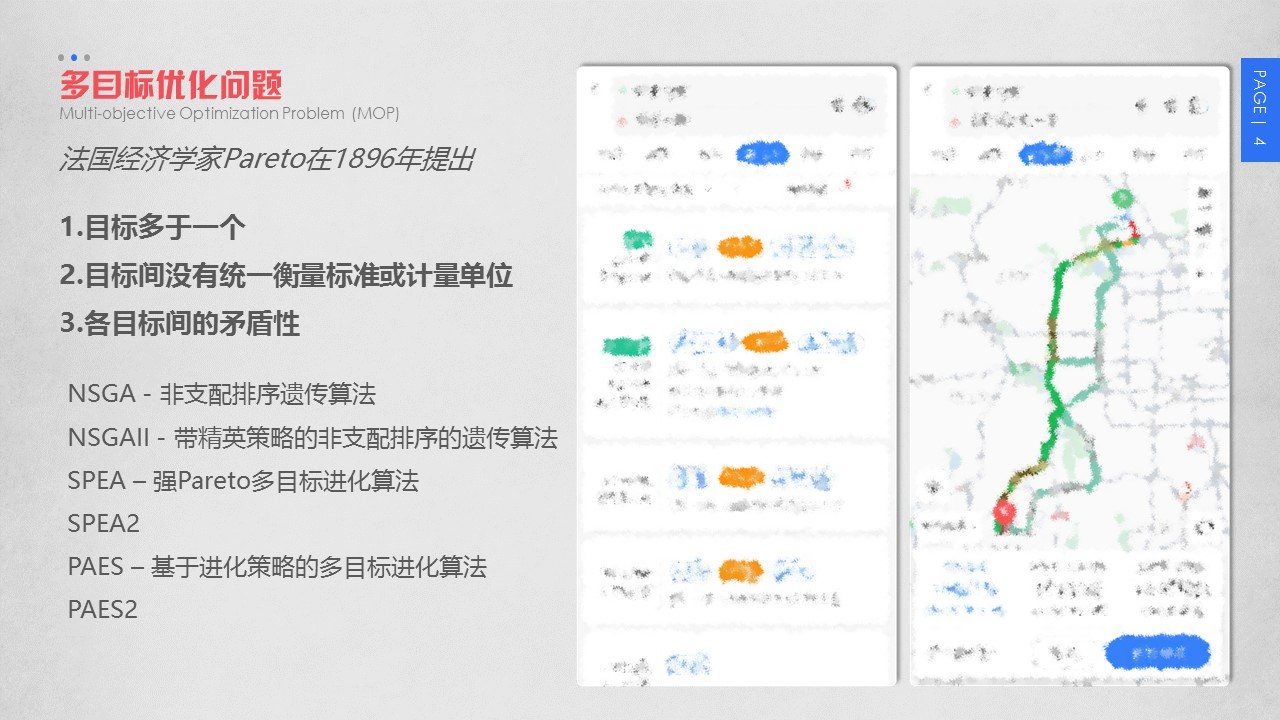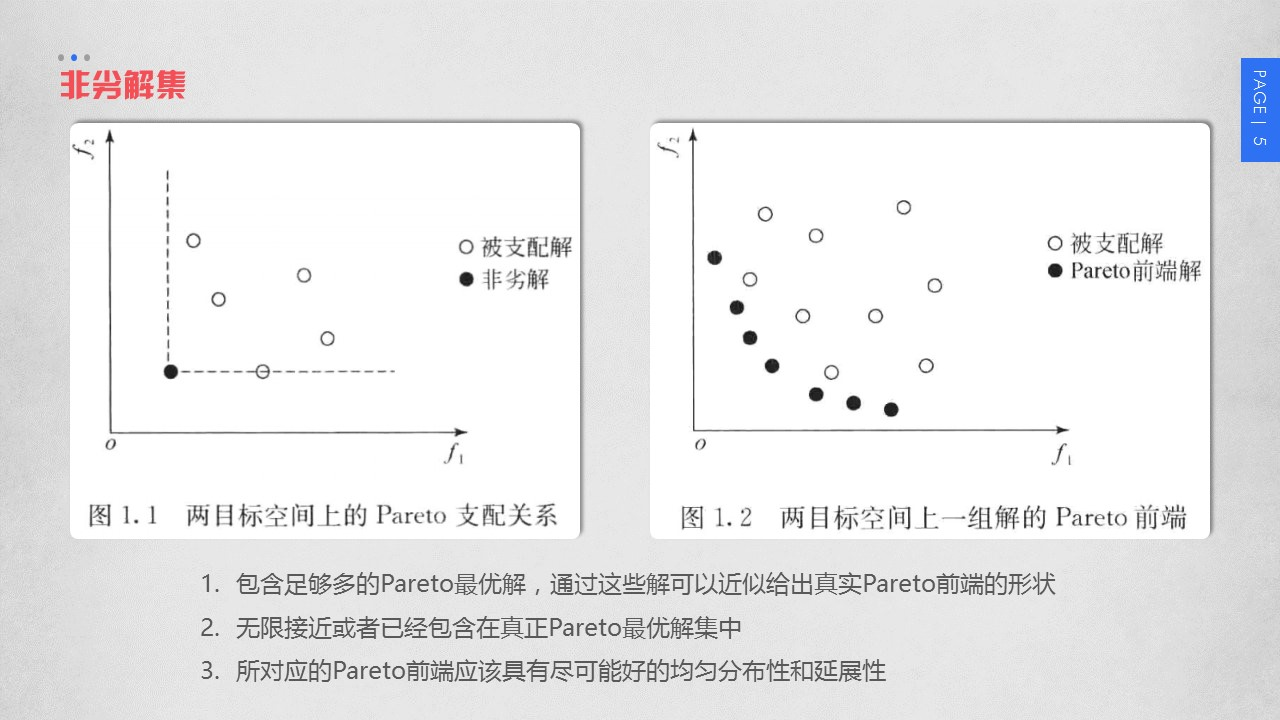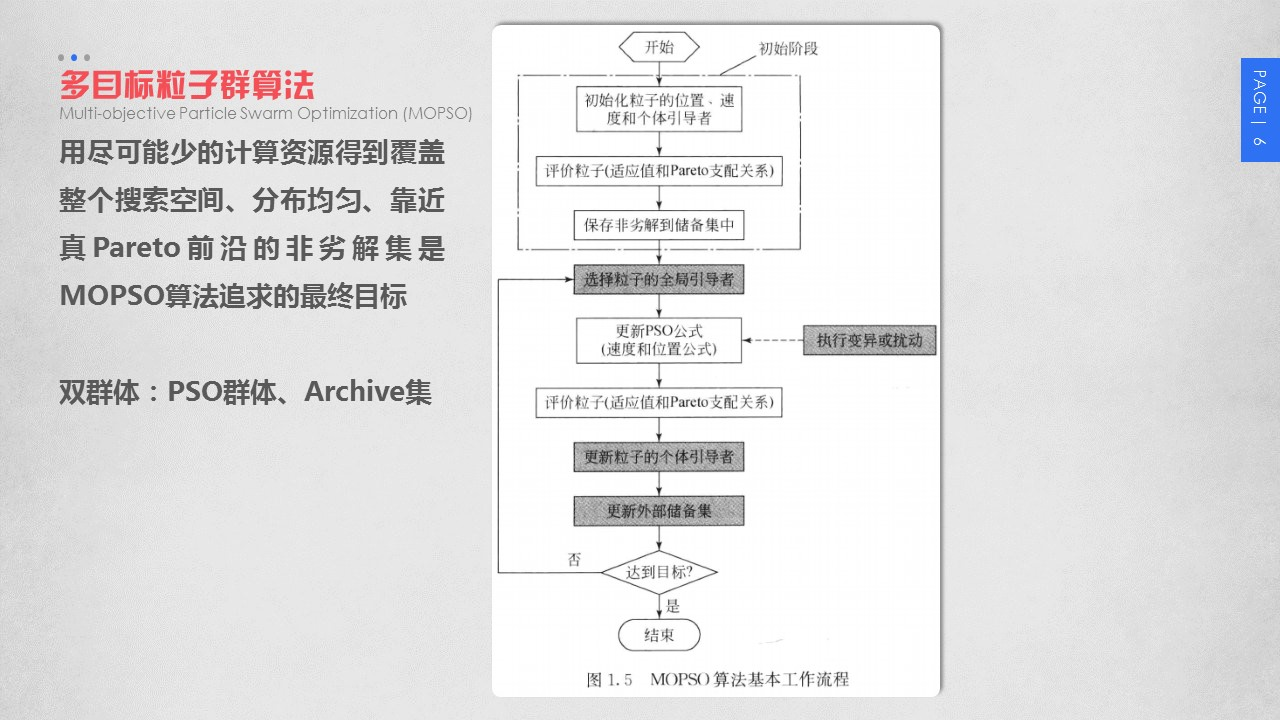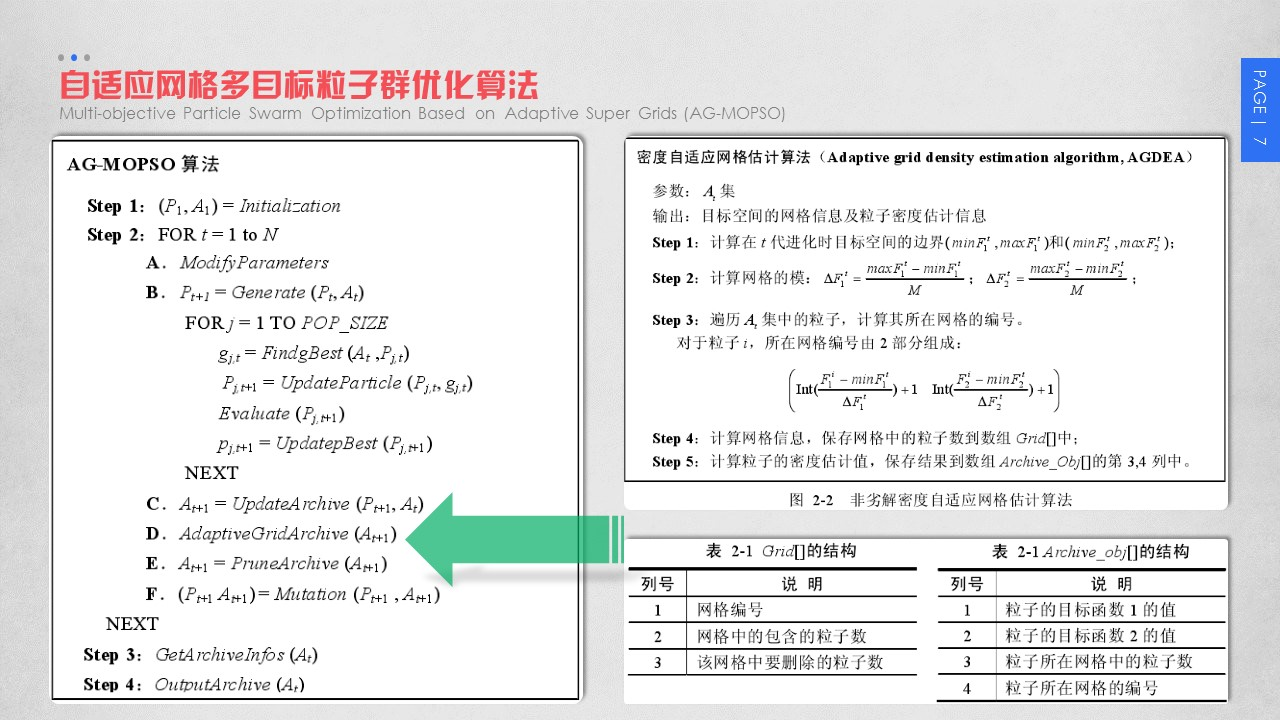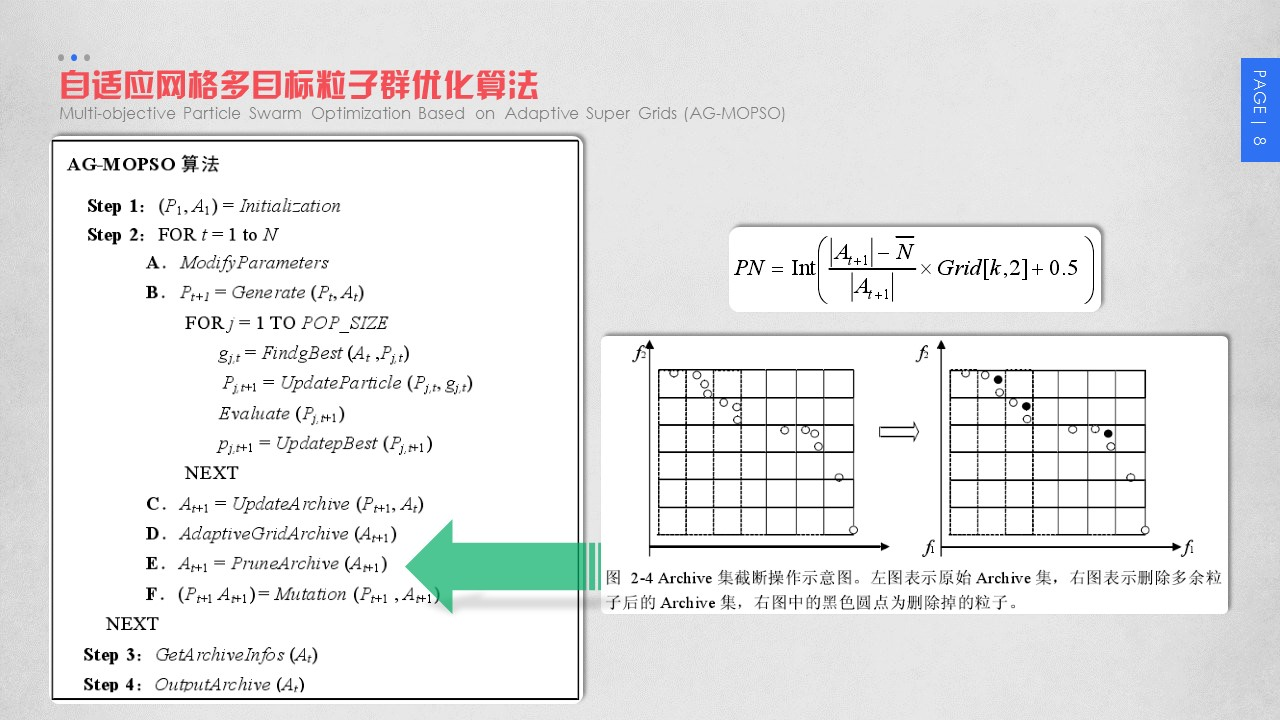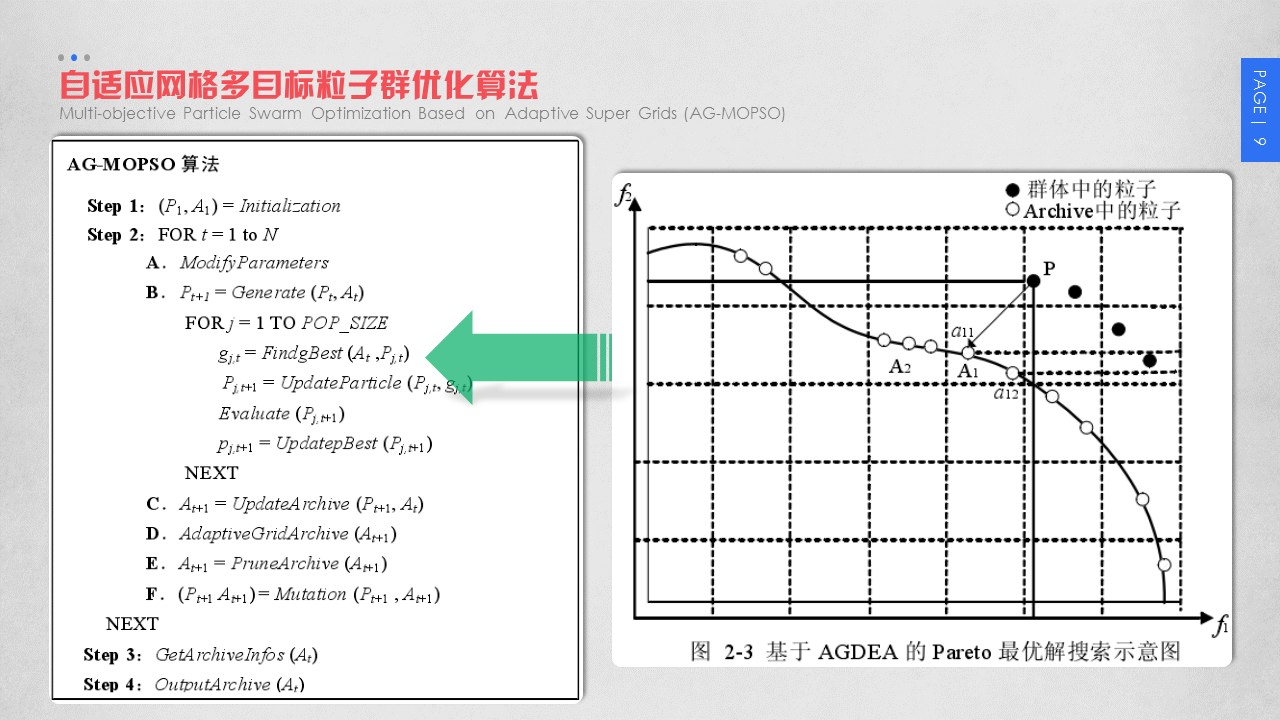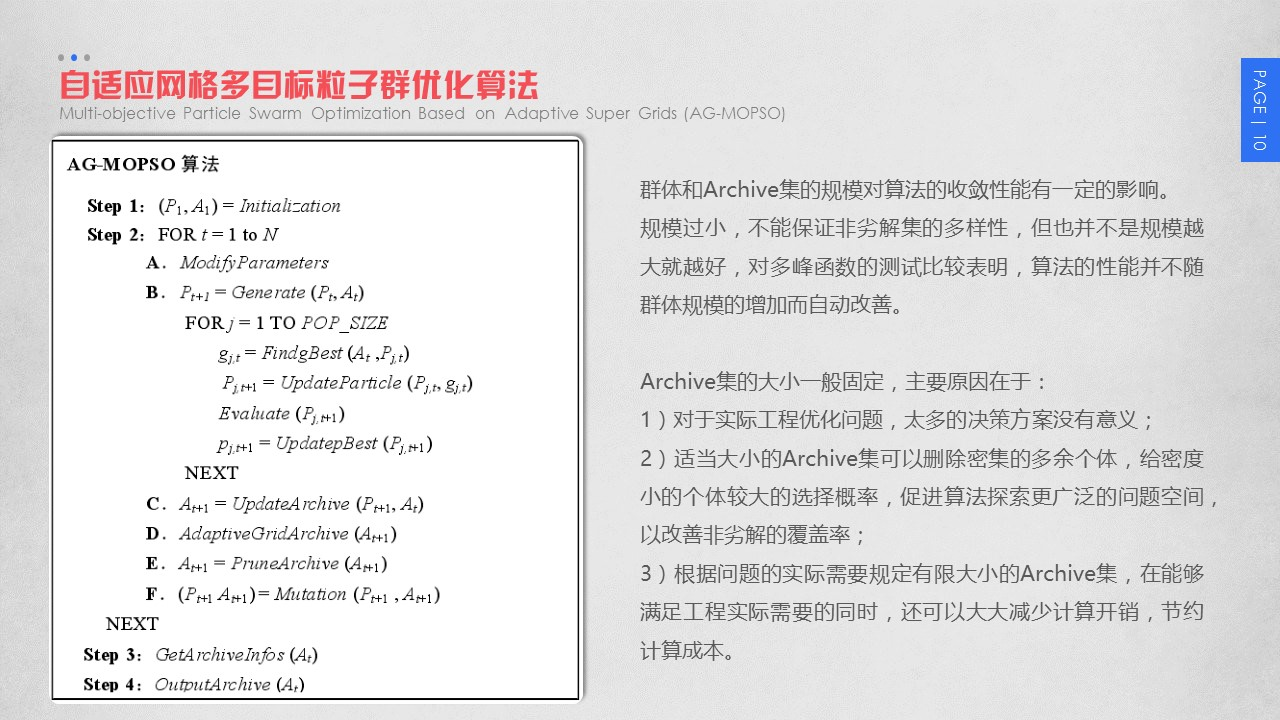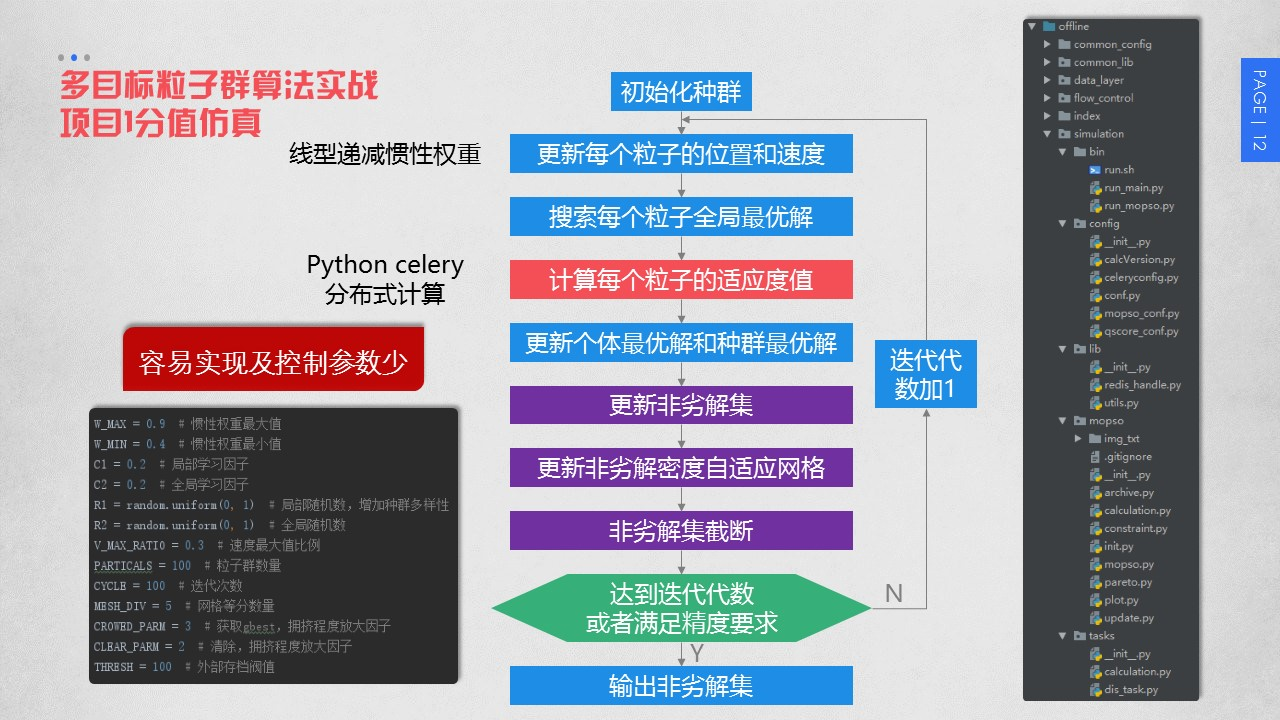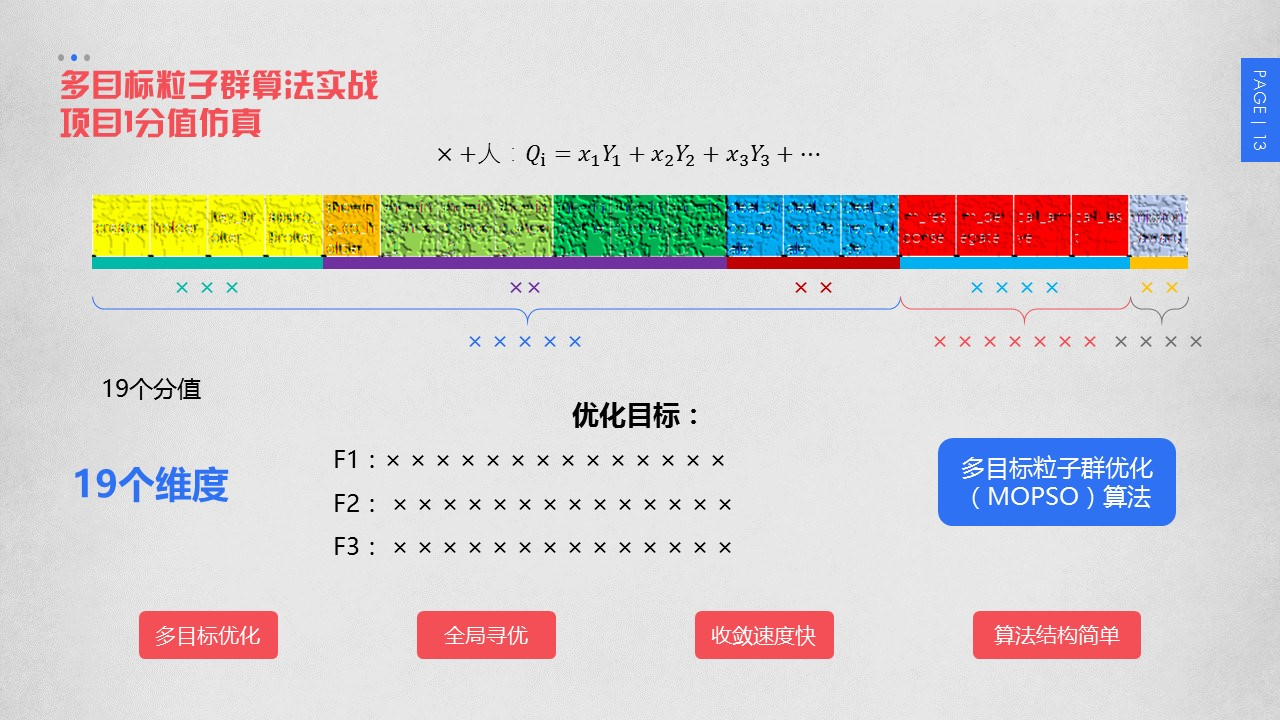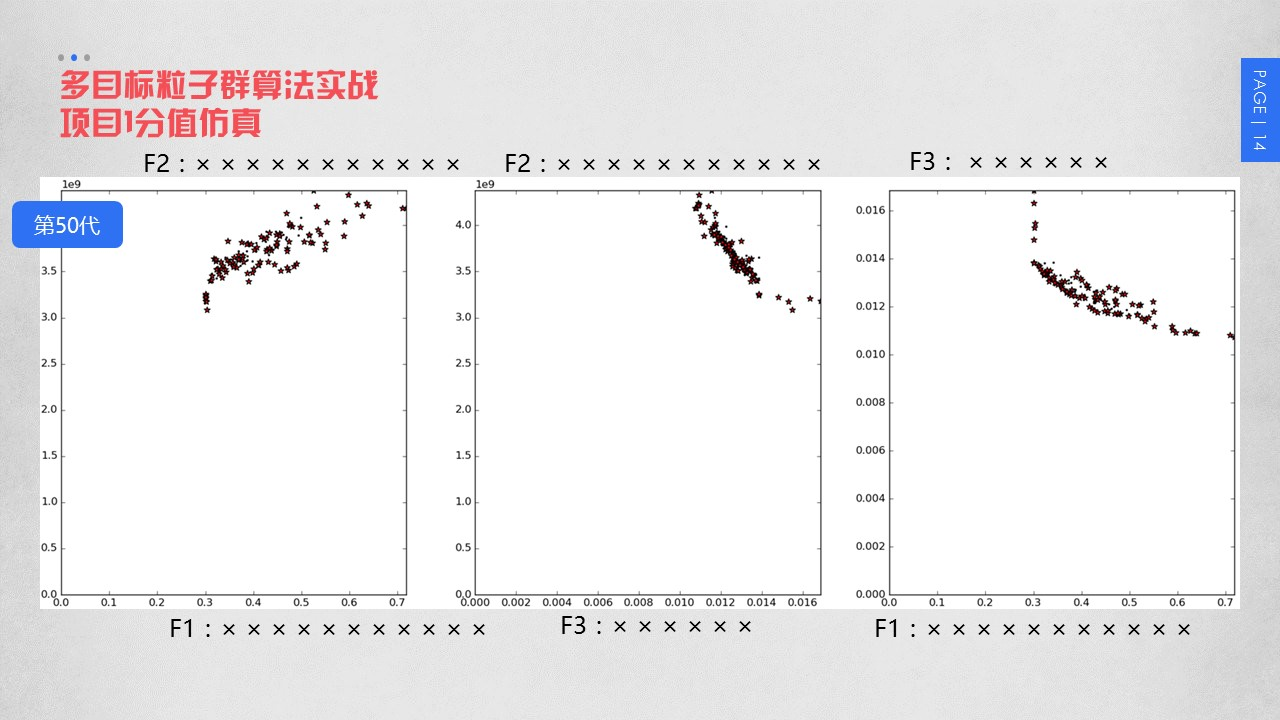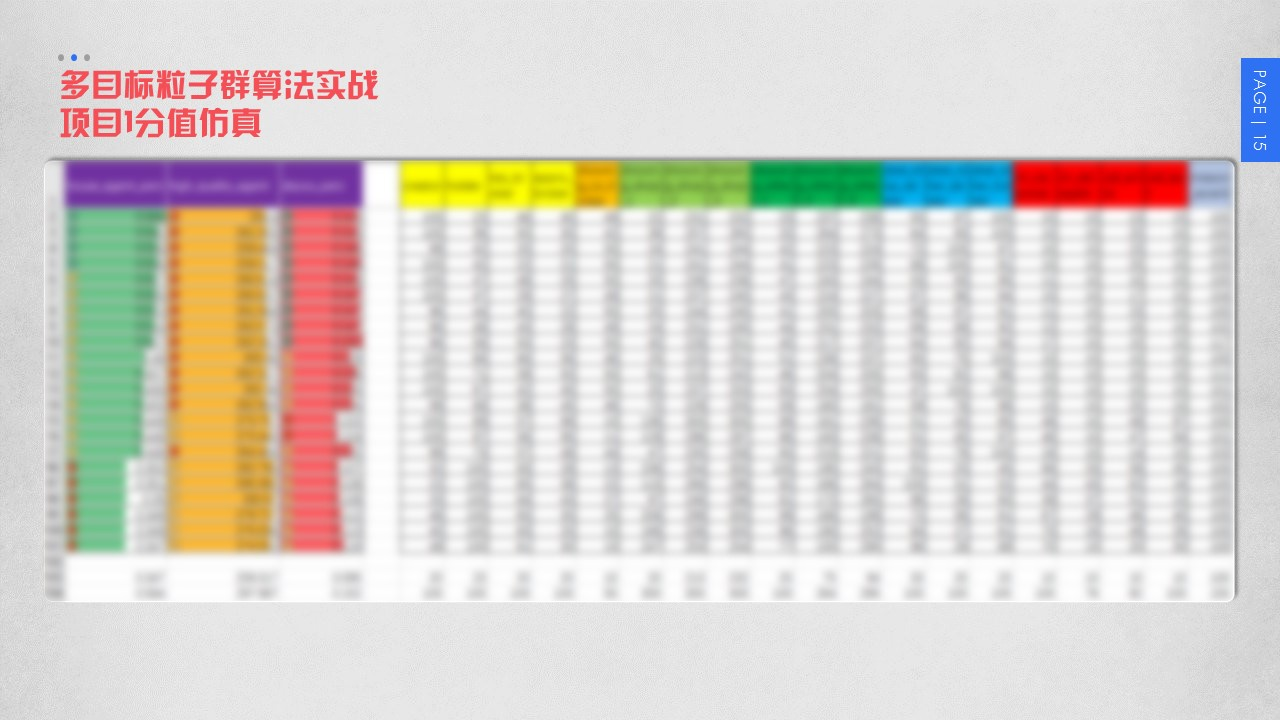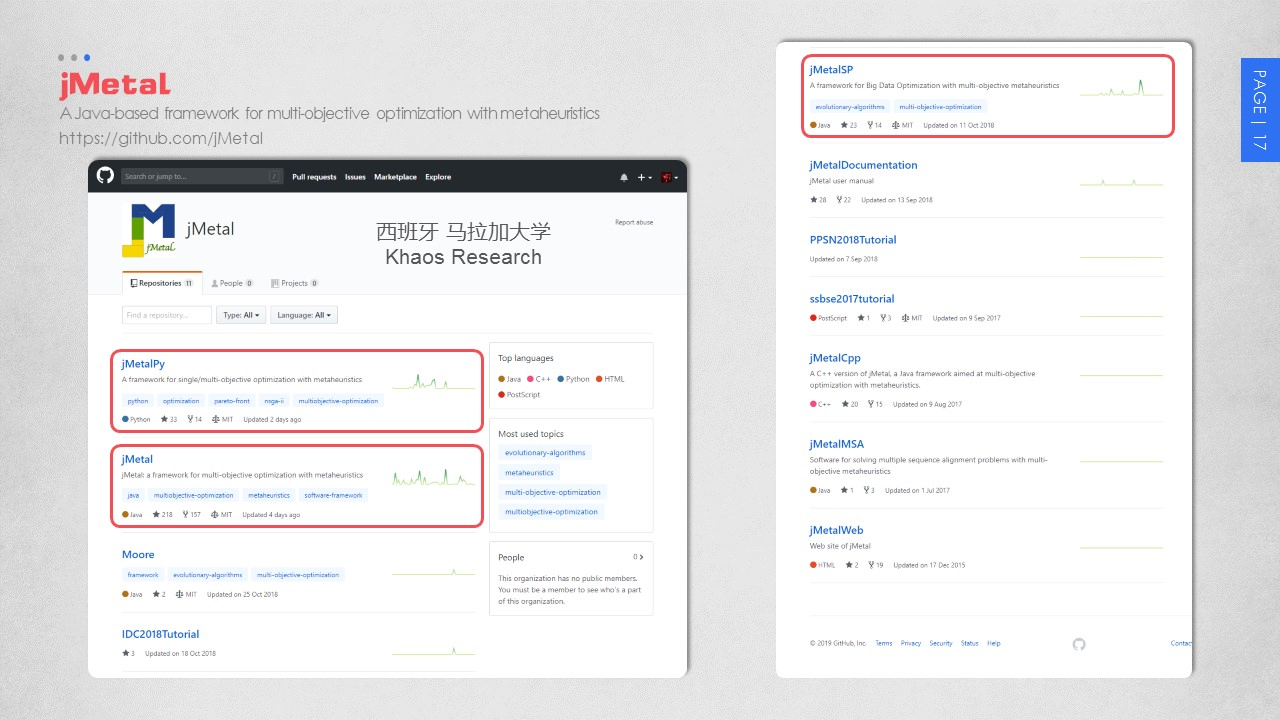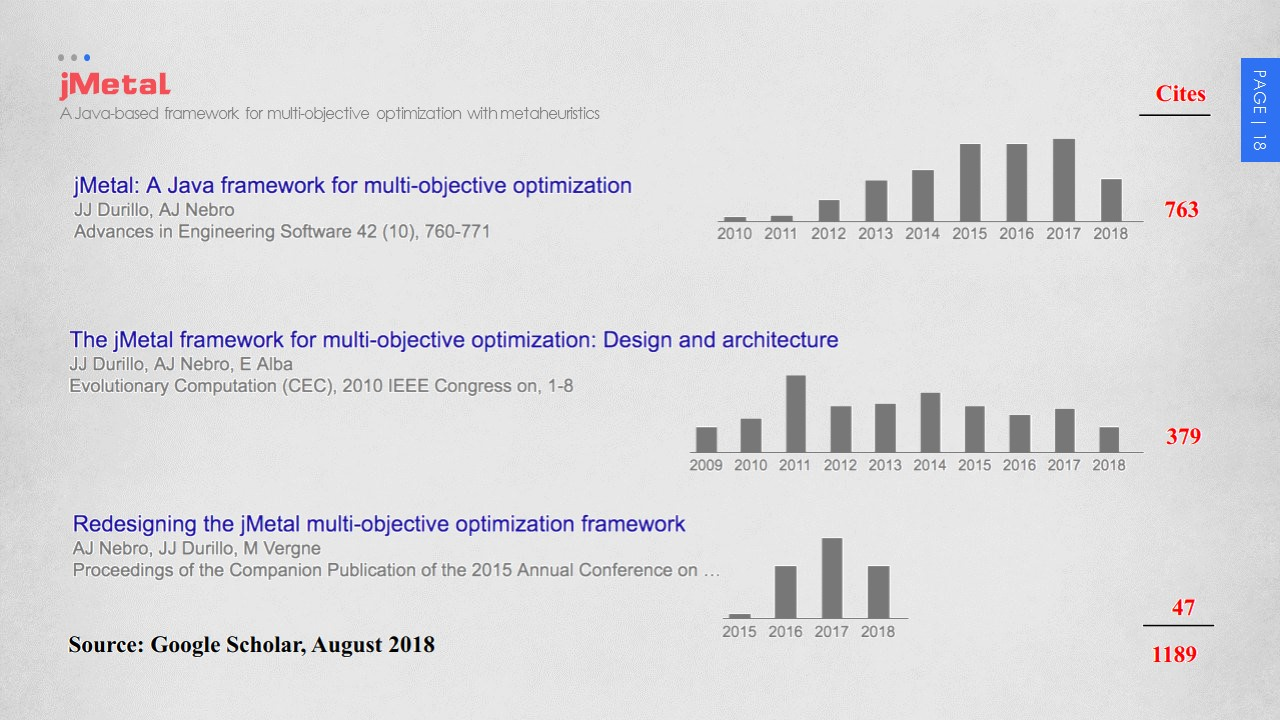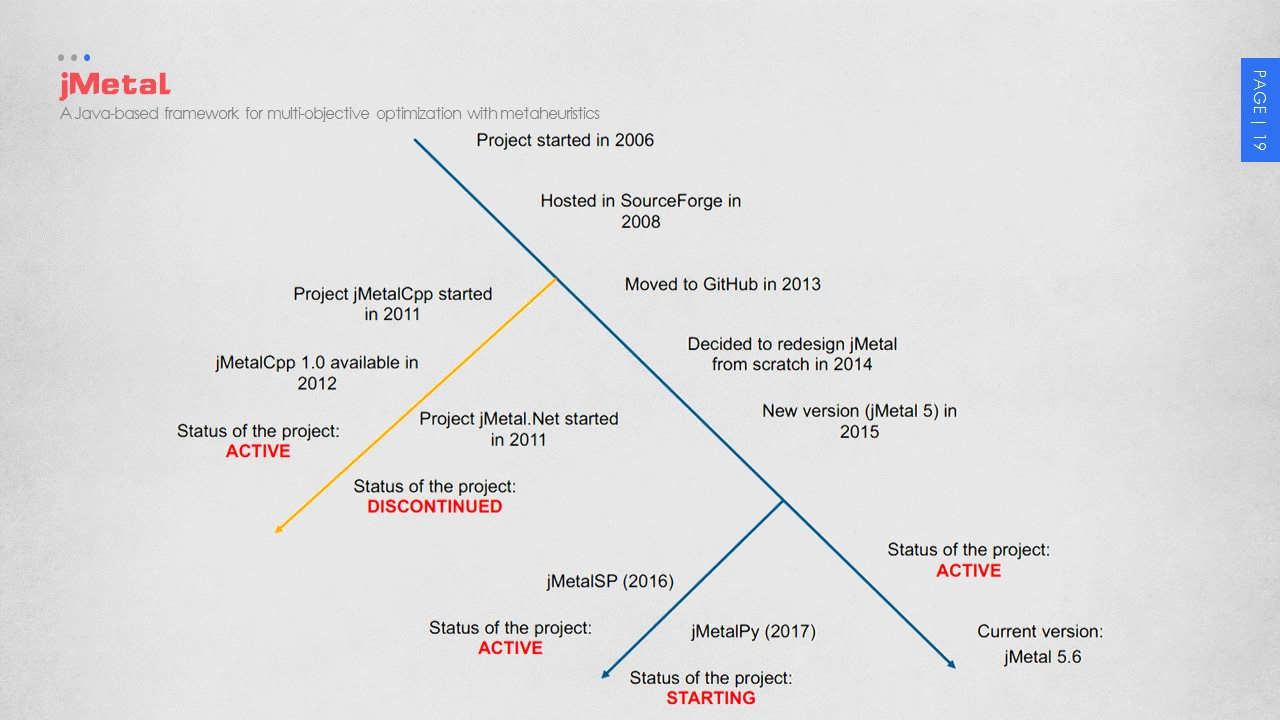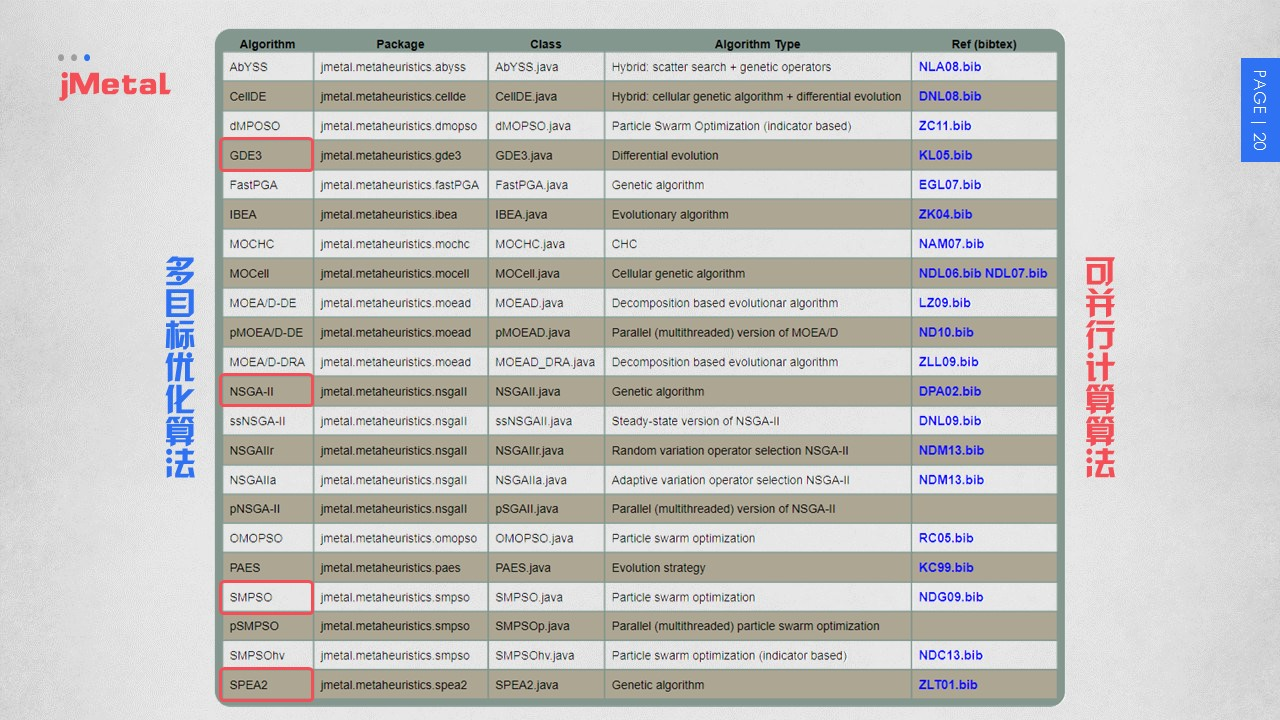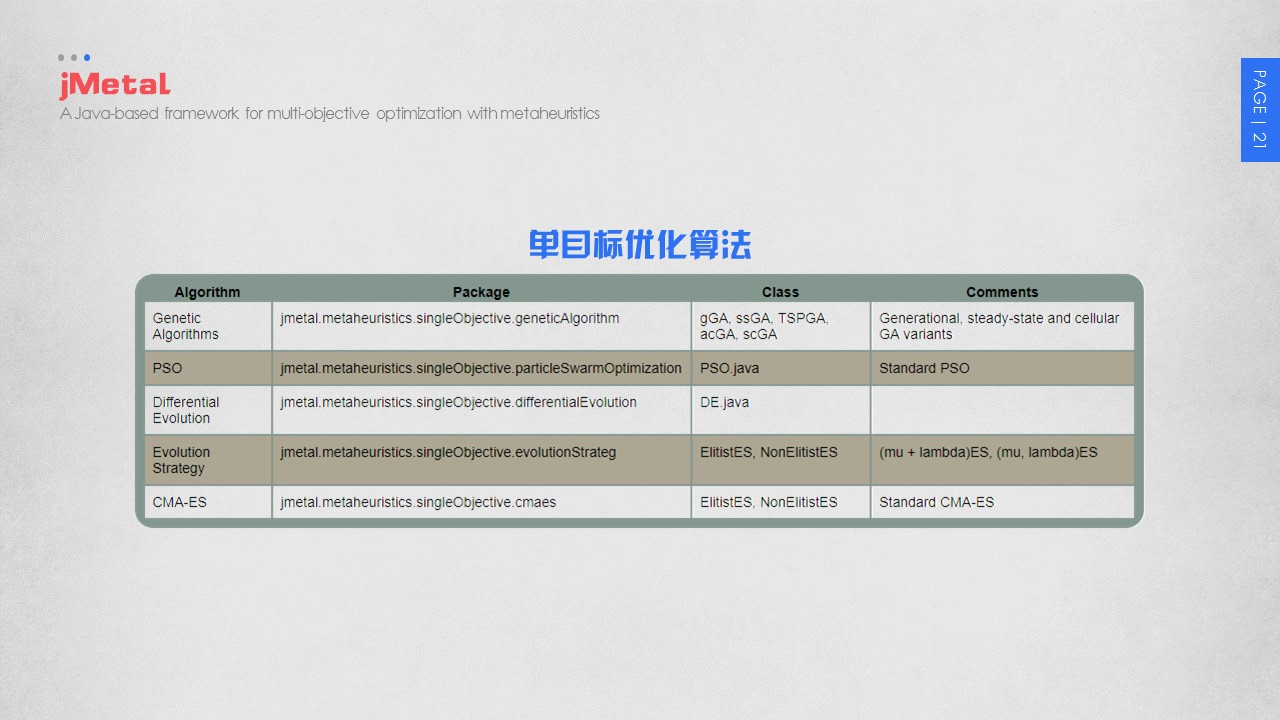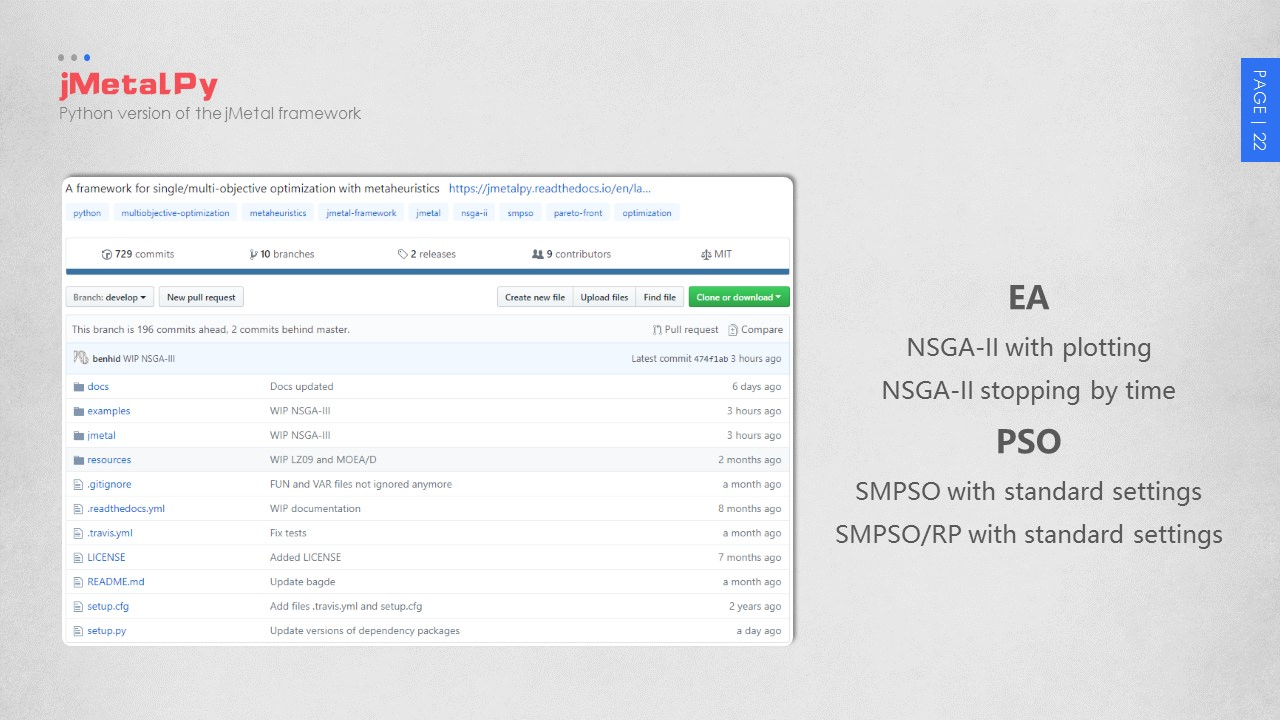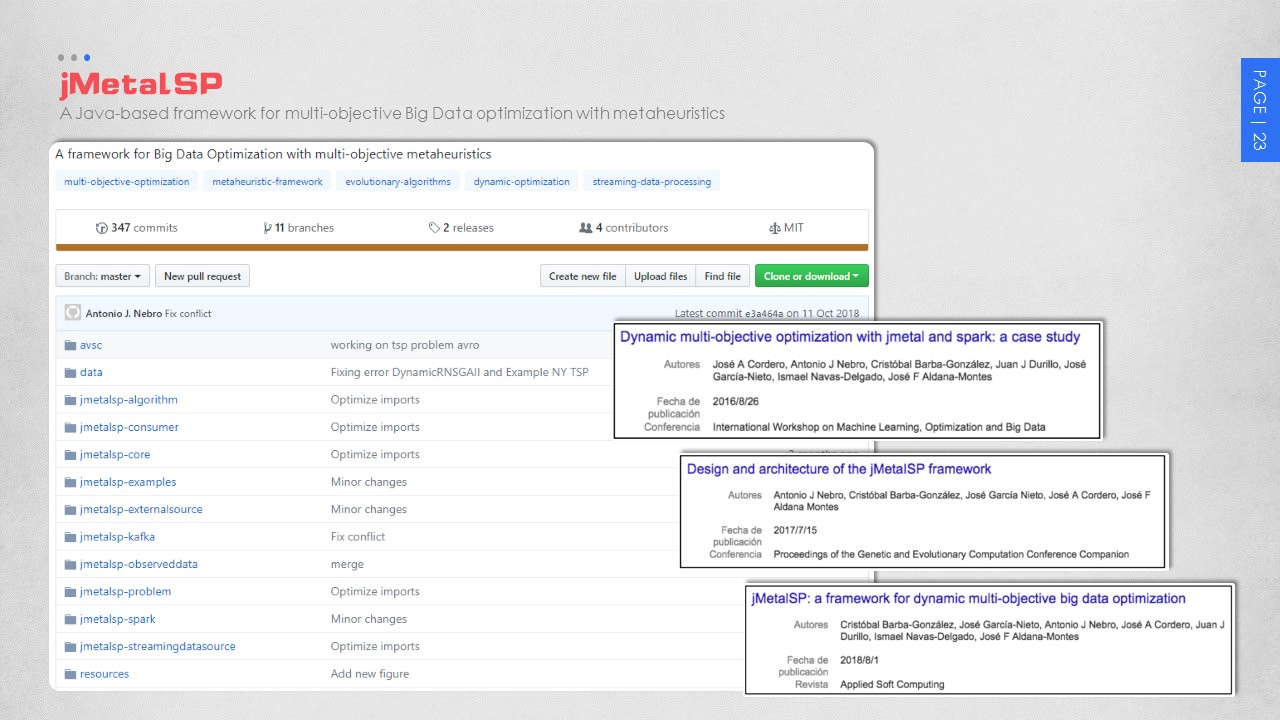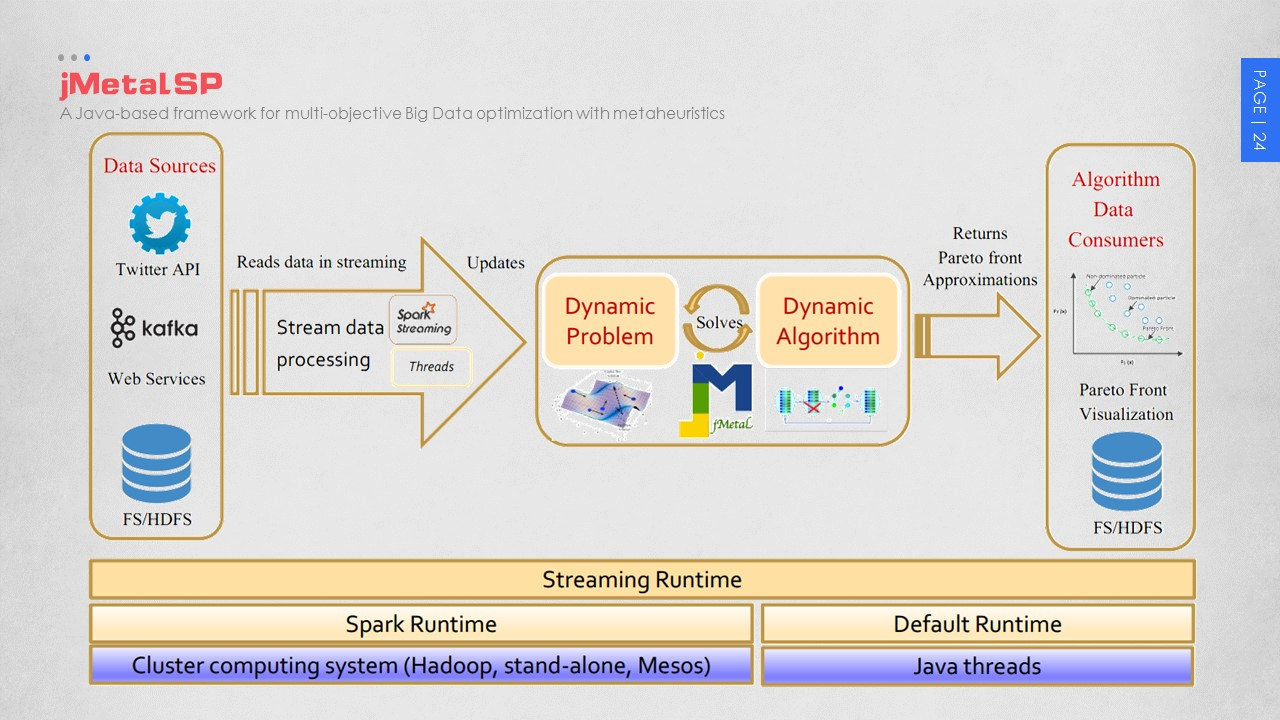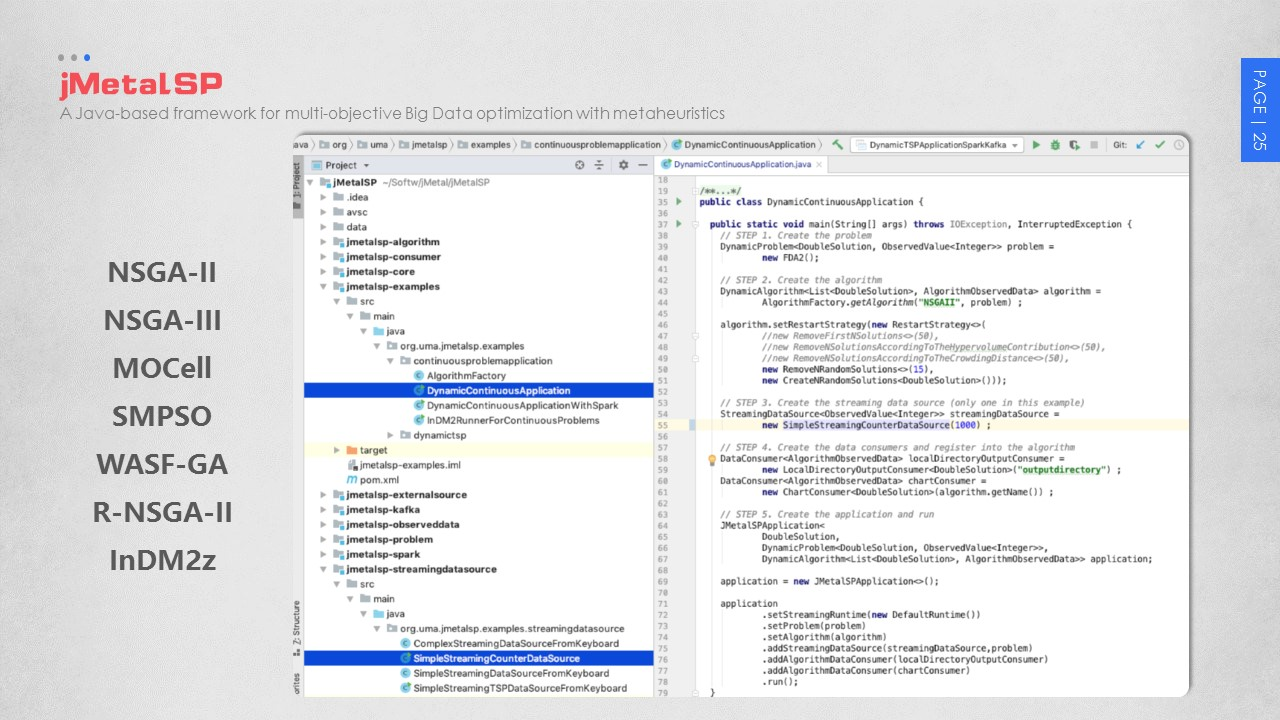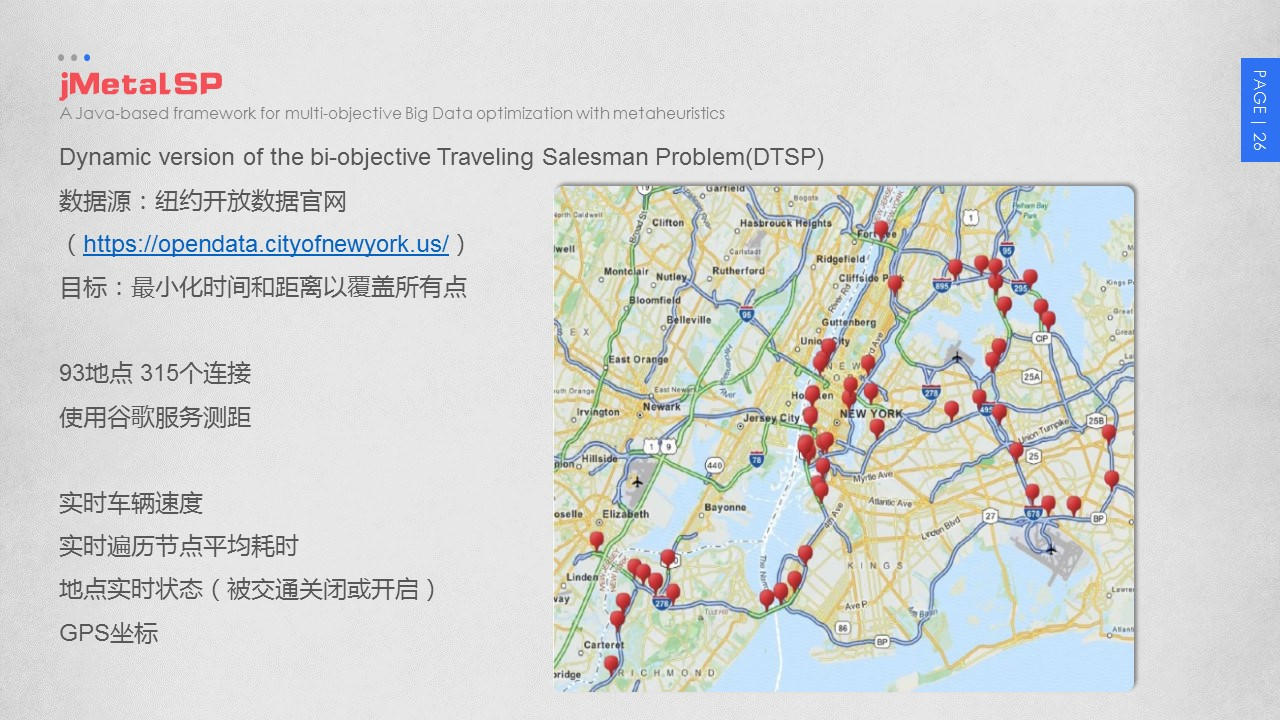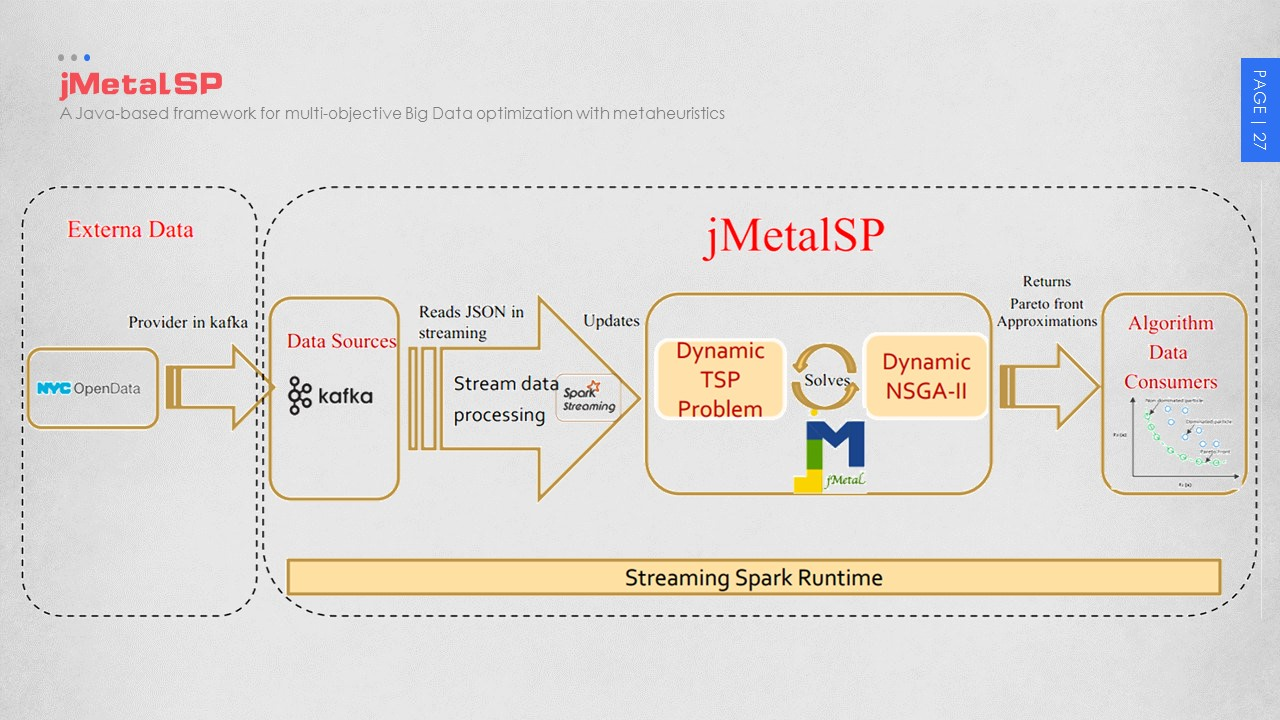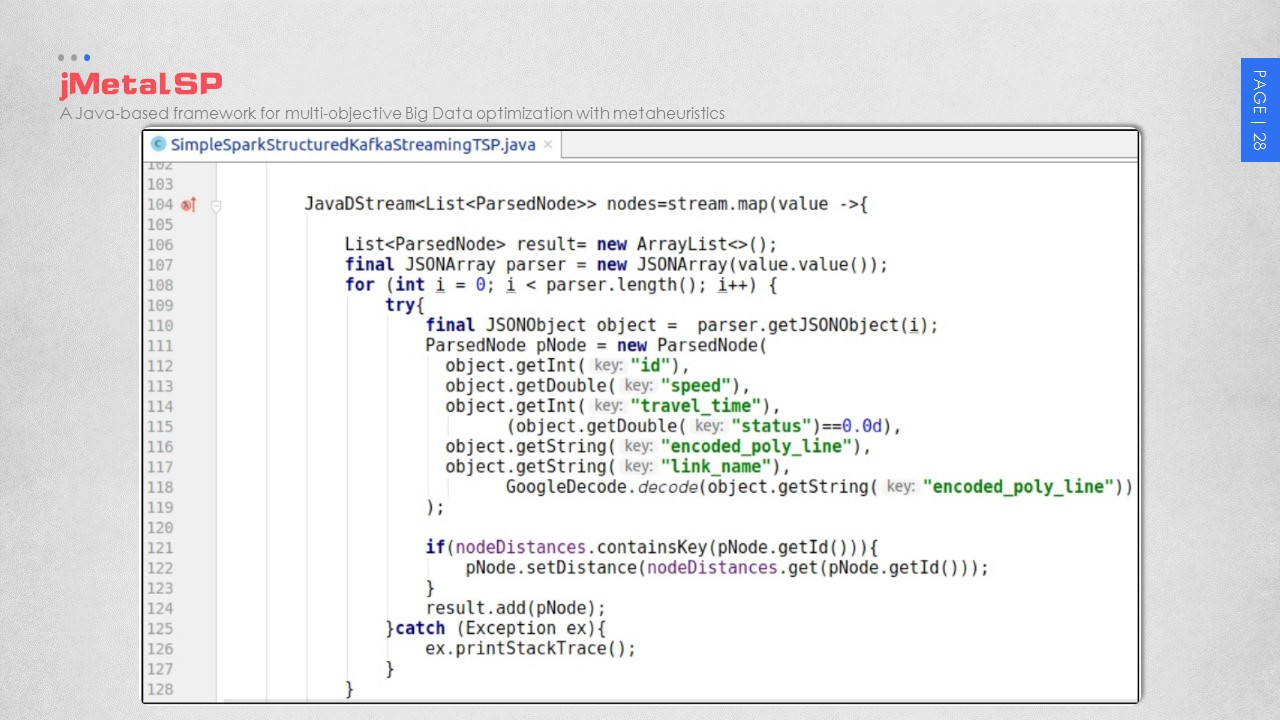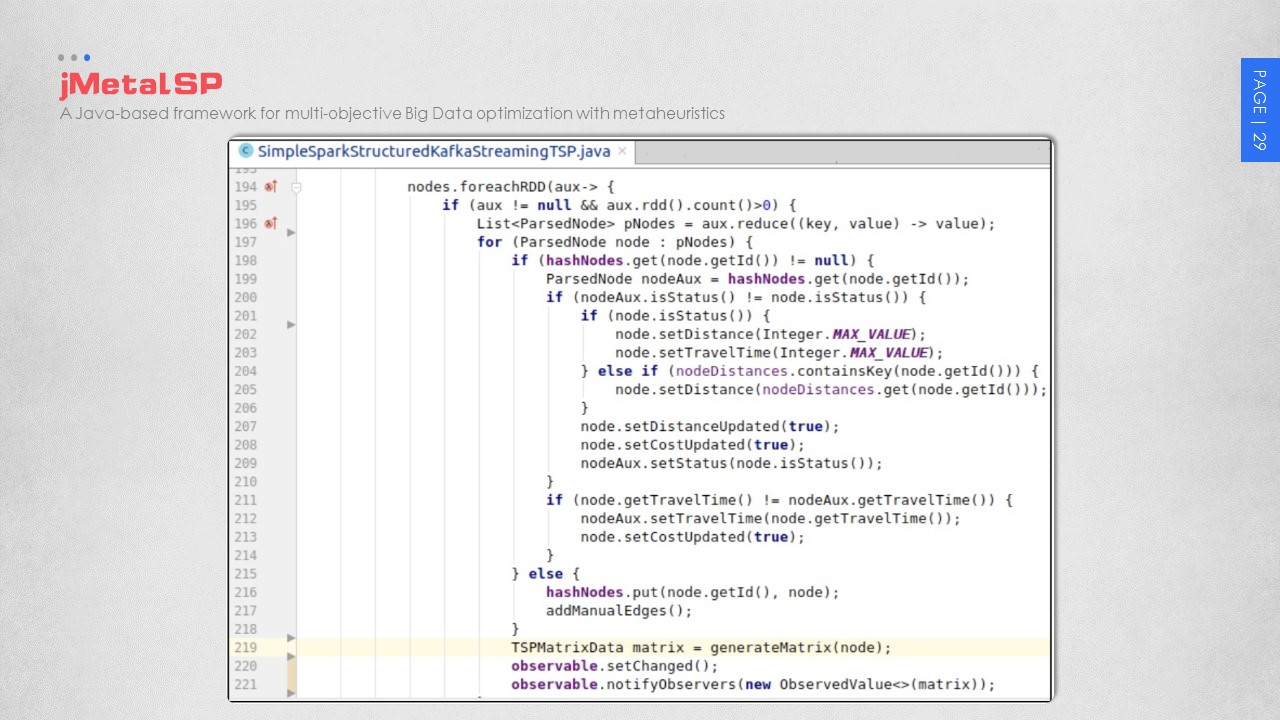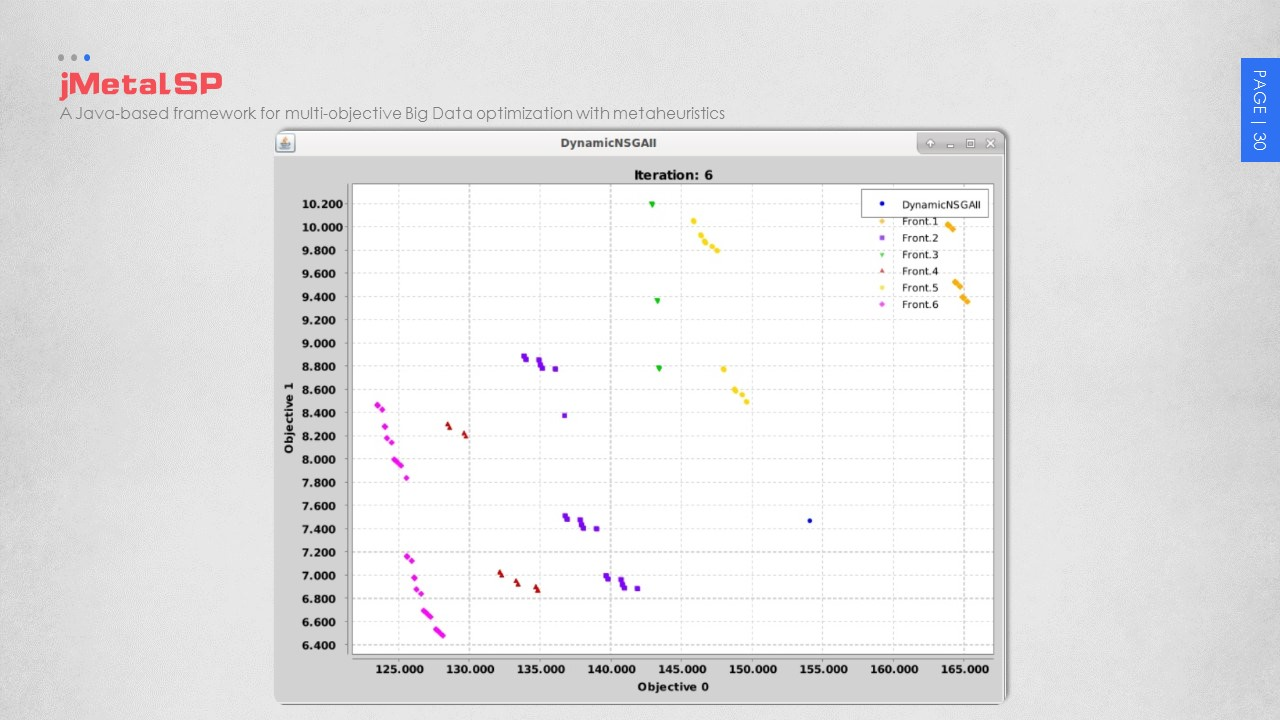--------------------------文档信息-------------------------- 版权声明：本文为博主原创文章，未经博主允许不得转载 署名(BY) ：dkjkls（dkj卡洛斯） 文章出处：http://blog.csdn.net/dkjkls
展开全文jMetal MOPSO
• 本代码为NSGA2的python版，论文原文复现结果，实验文档和matlab见之前的资源。nsga2
• 基于NSGA-II的多目标优化算法及论文，算法代码可运行，论文已在cscwd2018会议中发表
• 现在就进入正题吧，这个是一个多目标函数优化算法多目标函数优化有一种方法是，假如现在有n个目标函数fi,首先将每个目标函数乘以一个适当的参数alfai，再将所有的目标函数加起来，得到一个目标函数。这就将多目标...
•NSGAII
• 它实现了基于差分进化 (DE) 算法的基本多目标优化算法： “Storn, R., Price, K., 1997。差分进化：一种简单有效的连续空间全局优化启发式方法。全球优化杂志 11, 341 – 359。” 当一个目标被优化时，标准 DE ...matlab
• 最初，多目标优化问题→通过加权等方式转化为单目标问题→用数学规划求解。 这样每次只能得到一种权值下的最优解。而且MOP的目标函数、约束函数可能是非线性、不可谓、不连续的，传统的数学规划效率低，并且它们...
最初，多目标优化问题→通过加权等方式转化为单目标问题→用数学规划求解。
这样每次只能得到一种权值下的最优解。而且MOP的目标函数、约束函数可能是非线性、不可谓、不连续的，传统的数学规划效率低，并且它们对于权值或目标给定的次序比较敏感。
进化算法：通过代与代之间维持由潜在解组成的种群来实现全局搜索。

第一代EMO：采用基于非支配排序的个体选择方法和基于适应度共享机制的种群多样性保留策略。代表：NSGA。
采用基于非支配排序的思想选择较优个体时需要花费大量的计算量，并且选择效率比较低。
第二代EMO：以精英保留机制为特征。代表：NSGA-II。
在求解高维问题时多样性损失严重。
第三代EMO：多种新框架：
基于粒子群优化：PSO基于免疫算法：NNIA基于分解：MOEA/D（传统数学规划方法结合进化算法）

根据不同的选择机制，EMO分为三类：
基于Pareto支配：NSGA-II、SPEA2基于指标（比如通过使用HV指标进行选择较优个体保留，较劣个体删除）：IBEA基于分解：MOEA/D

第一代EMO：
NSGA：非支配解首先被确定。然后被分配一个很大的虚拟适应度值。为了保持种群的多样性,这些非支配解用它们的虚拟适应度值进行共享。这些非支配个体暂时不予考虑。 从余下的种群中确定第2 批非支配个体， 然后它们被分配一个比先前非支配个体共享后最小适应度值还要小的应拟适应度值。这些非支配个体也暂时不予考虑， 从余下的种群中确定第3 批非支配个体。该过程一直持续到整个种群都被划分为若干等级为止NSGA采用比例选择来复制出新一代.NSGA 的计算复杂度为，其中声是目标个数万是种群大小.其计算复杂度较高， 而且需要预先确定共享参数。NPGA ：设计了基于Pareto支配关系的锦标赛选择机制。具体思想如下：随机地从进化种群中选择两个个体，再随机地从进化群体中选取一个比较集， 如果只有其中1 个个体不受比较集的支配， 则这个个体将被选中进入下一代；当它们全部支配或全部被支配于该比较集时，采用小生境技术实现共享来选取其中之一进入下一代。算法选取共享适应值大的个体进入下一代.该算法中，小生境半径的选取和调整比较困难，还要选择一个合适的比较集的规模。
第一代进化多目标优化算法以基于非支配排序的选择和基于共享函数的多样性保持为其主要特点。在第一代进化多目标优化的发展期间，一些亟需解决的问题也凸显出来。首先， 能否找到替代小生境(共享函数)的方法来保持种群的多样性。适应度共享是针对多峰函数优化提出来的，通常需要关于有限峰数量的先验知识和解空间小生境均匀分布的假设。对于多目标优化问题，同样需要确定共享半径的先验信息，其计算复杂度为种群大小的平方。

第二代EMO：
NSGA-II：是2002年Deb 等人对其算法NSGA的改进，它是迄今为止最优秀的进化多目标优化算法之一。相对于NSGA而言,NSGA-II具有以下优点：① 新的基于分级的快速非支配解排序方法将计算复杂度由降到，其中，m 表示目标函数的数目、N 表示种群中个体的数目。② 为了标定快速非支配排序后同级中不同元素的适应度值，同时使当前Pareto前沿面中的个体能够扩展到整个Pareto前沿面，并尽可能地均匀遍布。该算法提出了拥挤距离的概念，采用拥挤距离比较算子代替NSGA中的适值度共享方法，拥挤距离的时间复杂度为。③ 引入了精英保留机制，经选择后参加繁殖的个体所产生的后代与其父代个体共同竞争来产生下一代种群，因此有利于保持优良的个体,提高种群的整体进化水平。
第二代EMO以精英保留策略为主要特征，并且大多数算法不再以适应度共享的小生境技术作为保持种群多样性的手段，一些更好的策略被提出来，比如基于聚类的方法、荃于拥挤距离的方法、墓于空间超格的方法。

当前EMO研究热点：
基于粒子群优化的多目标优化：PSO是1995年由提出的群智能优化算法,它将种群中每个个体看成搜索空间中的一个没有体积和质量的粒子.这些粒子在搜索空间中以一定的速度飞行,其速度根据其本身的飞行经验和整个种群的飞行经验进行动态调整.优点在于流程简单易实现,算法参数简洁, 无需复杂的调整, 因此, 从提出至今, 已被迅速用于遗传算法原有的一些应用领域.墓于人工免疫系统的多目标优化：是受免疫学启发, 模拟免疫学功能、原理和模型来解决复杂问题的自适应系统, 已被成功用于异常检测、计算机安全、数据挖掘、优化等领域。NNIA模拟了免疫响应中多样性抗体共生 、少数抗体激活的现象, 通过一种基于非支配邻域的个体选择方法, 只选择少数相对孤立的非支配个体作为活性抗体,根据活性抗体的拥挤程度进行比例克隆复制, 对克隆后的抗体群采用了有别于G A 的重组操作和变异操作, 以此加强对当前P aer ot 前沿面中较稀疏区域的搜索. NNIA是一种非常有效的EMO算法。当目标个数达到9 时, 对于较困难的DTLZ 问题,NNIA仍能得到较为令人满意的性能, 显示了该方法在求解高维多目标优化问题时具有很大的优势基于分布估计算法的多目标优化：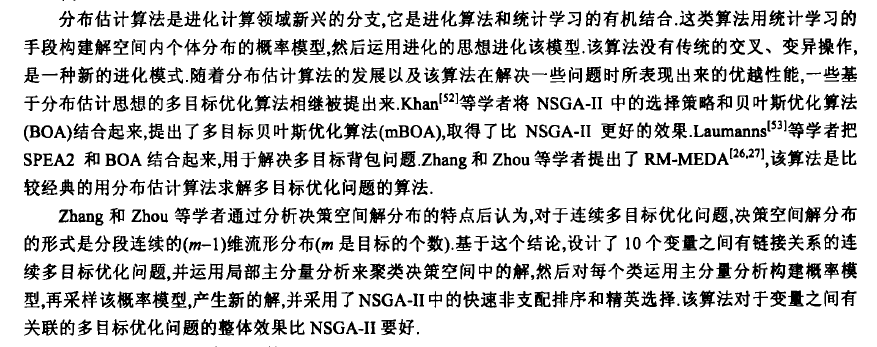基于分解的多目标进化算法：将多目标优化问题转换为单目标优化问题是用数学规划方法求解多目标优化问题的基本策略, 典型的转 换方法包括权重和法、切比雪夫法、边界交叉法等.该算法将通近整个Pareto前沿面的问题分解为一定数量的单目标优化问题,然后用进化算法同时求解这些单目标优化问题. 算法维持一个由每个子问题的当前最优解组成的种群, 子问题之间的近邻关系定义为子问题权重向量之间的距离, 每个子问题的优化过程通过与其近邻子问题之间的进化操作来完成.该算法成功地将数学规划中常用的分解方法引入到进化多目标领域,而且可以直接采用进化算法求解单目标优化问题时的适应度分配和多样性保持策略. 将数学规划方法与进化算法相结合是求解多目标优化问题的一种有效方法, 为进化多目标优化提供了一种新思路。

测试问题：
ZDT问题由6 个具有不同性质的两目标优化问题组成, 其PF已知, 是目前采用得最多的测试问题之一。
DTLZ问题能够扩展到任意多个目标, 从而能够很好地扩展为高维多目标优化问题，也是目前采用得最多的测试问题之一。但是，ZDT问题和DTLZ问题也具有明显的缺点，如目标函数缺乏平坦区域、缺少连续空间上的骗问题、PF和定义域空间过于简单等等。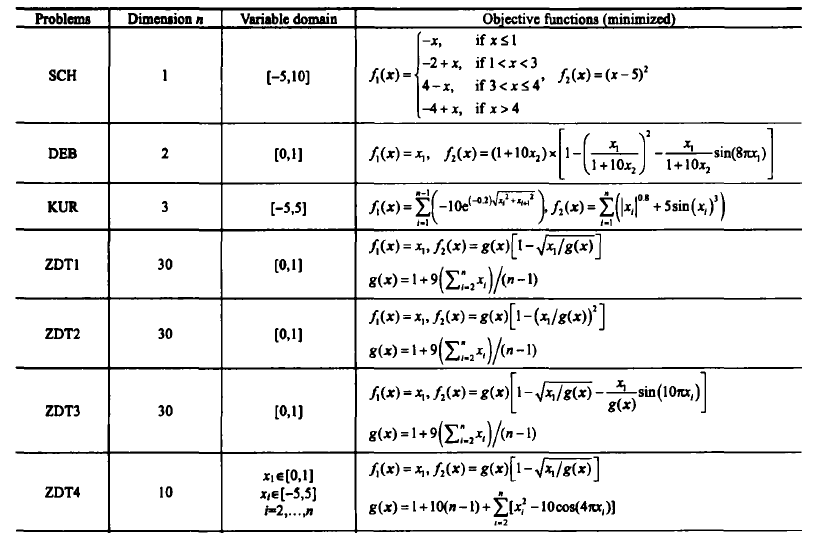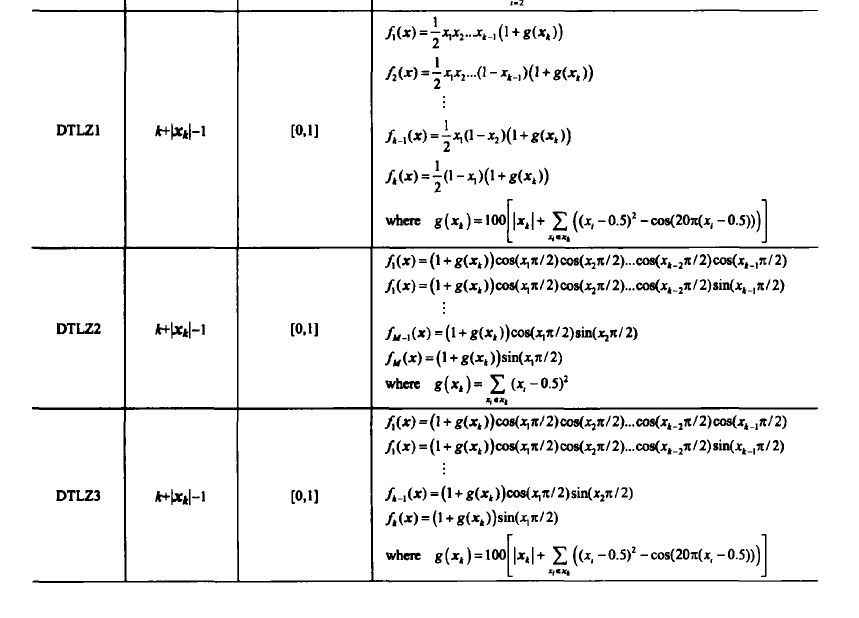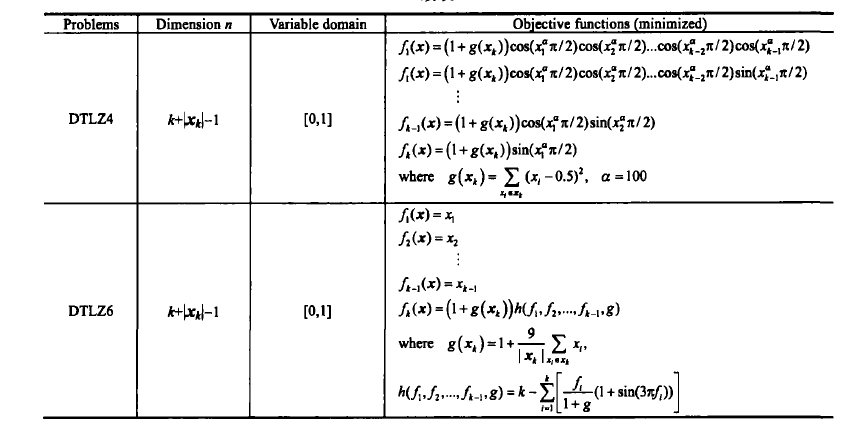指标：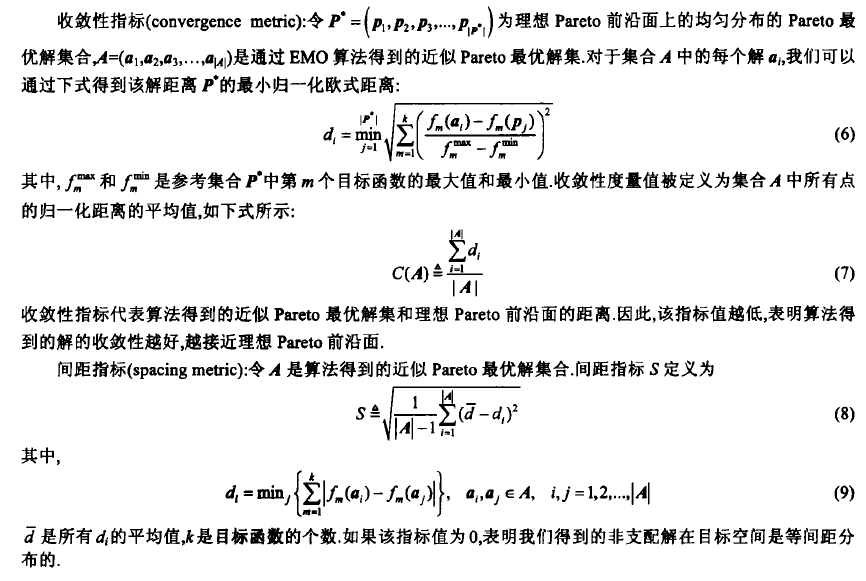展开全文• 多目标优化算法（一）：知识储备 算法小白第一次记笔记，多多指教！ 注：我最近在研读向毅博士的毕业论文，以下知识点来自其博士论文，但是也有我自己的理解与总结。在此附上论文的百度学术链接：...
算法小白第一次记笔记，多多指教！ 注：我最近在研读向毅博士的毕业论文，以下知识点来自其博士论文，但是也有我自己的理解与总结。在此附上论文的百度学术链接：https://xueshu.baidu.com/usercenter/paper/show?paperid=1g5806j0910a00p0rd3g0ax0r5437805&site=xueshu_se
目录
智能优化算法的优势优化问题的分类高维多目标演化算法的分类（MaOEAs）多目标优化问题基本定义多目标测试问题集多目标算法性能评价指标
智能优化算法的优势
与数学方法相比，演化算法和基于群集智能算法的优势为：
不要求当前问题具有较好的解析性质，如可导，连续等；可处理大规模，甚至极为复杂的搜索空间；基于种群（或群体）特性，使算法单次运行即可产生真实帕累托前沿近似解集。
优化问题的分类
在科学研究及工程实践中，人们往往会遇到各种优化问题。一般来说，这些优化问题被分为单目标优化问题和多目标优化问题。
单目标优化问题(Single-objective optimization problem,SOPs) :待优化的目标只有一个；一个问题对应一个最优解。多目标优化问题(Multi-objective optimization problem,MOPs):至少存在两个目标，且各目标之间往往冲突，即一个目标的改善通常会导致至少一个其它目标的退化。当目标个数超过3，称为高维多目标优化问题（Many-objective optimization problem,MaOPs）
高维多目标演化算法的分类（MaOEAs）
基于改进的Pareto支配算法：比较个体时考虑目标真实值；比较个体时考虑各目标比较的优劣数。缺点是涉及问题相关控制参数。基于改进多样性维护方法的算法：代表性算法如GrEA（引入3个准则维护多样性），SDE（基于转换的密度评估策略）。缺点是过分强调多样性可能会对收敛性造成影响。基于分解的算法：：常采用一组权向量（或参考方向）和特定成就标量函数（ASF）将多目标问题转化为一系列标量子问题。缺点是需在高维目标空间生成一组分布均匀的权向量。代表性算法框架是MOEA/D。基于性能指标的算法：常用的指标为HV，IGD，R2等。缺点是计算效率低下。其它类别的高维多目标演化算法：基于新算法框架的算法，基于目标降维的算法，基于用户偏好的算法等。
多目标优化问题基本定义
Pareto最优：在比较解的优劣时，单目标优化直接根据适应度值大小比较不同解的优劣，但是多目标优化中目标值不只一个，根据某一个目标值决定优劣关系是不合理的，常用的比较方法是非支配排序法。  Pareto支配：图中给出一个双目标最小化的例子，B和A比较时，B的两个目标值都比A好，因此B肯定比A好，则称B支配A；B和C比较时，B的第一个目标比C好，但是第二个目标比C差，此时无法判断B和C哪个更好，则称B和C是互不支配的关系。Pareto前沿：在一个种群中，存在一个不被其他任何个体支配的解集，这个解集在目标空间的映射称为Pareto前沿（PF）。Pareto解集：相应地，在决策空间称为帕累托解集（PS）。多目标测试问题集
为了验证进化多目标算法（EMO）的性能，学者们设计了众多测试问题。这些测试问题的设计主要参考以下性质： –决策变量和目标个数可调 –PS形状多样 –PF形状多样 –真实PS和PF已知 –等效最优解集个数可调 –具有全局和局部最优解集
常用的基准测试函数集
DTLZ（7个问题） –所有问题均可分 –可任意设定决策变量和优化目标的个数 –DTLZ1的PF是一个超平面 –DTLZ2是一个相对简单问题 –DTLZ3非常适合检验算法收敛性 –DTLZ4使得一般EMO算法难以获得分布广泛的最终解集 –DTLZ5,6是退化的曲面，可检测算法在高维目标空间搜索低维PF的能力 –DTLZ7可用于检测算法是否具备有效处理不连续PF的能力 文献：Deb K, Thiele L, Laumanns M, et al. Scalable test problems for evolutionary multiobjective optimization. Springer, 2005.  WFG（9个问题） –可任意设定决策变量和优化目标的个数 –大多数问题不可分 –引入更多问题特性：单峰/多峰，有偏/无偏，欺骗性/无欺骗性，凹状/凸状，退化/非退化等 文献：Huband S, Hingston P, Barone L, et al. Areview of multiobjective test problems and a scalable test problem toolkit. IEEE Transactions on Evolutionary Computation, 2006, 10(5):477–506.  DTLZ-1/WFG-1 –在原始DTLZ和WFG测试问题各目标函数前乘以（-1）即可，其目的在于获得反转的PF –该测试问题的主要目的在于测试EMO算法处理凸状PF的能力 文献：Ishibuchi H, Setoguchi Y, Masuda H, et al. Performance of Decomposition-Based Many-Objective Algorithms Strongly Depends on Pareto Front Shapes. IEEE Transactions on Evolutionary Computation, 2017, 21(2):169–190.  MMF suite –不可任意设定决策变量和优化目标的个数 –PF的几何形状为凸/凹/线性 –PS的几何形状为曲线段 –PS个数不可调 –函数比较简单 文献：J. J. Liang, W. Xu, C. Yue, K. Yu, H. Song, O. D. Crisalle, and B. Qu, “Multimodal Multiobjective Optimization with Diffierential Evolution,” Swarm and Evolutionary Computation, vol. 44, no. 2019, pp. 1028-1059, 2018.  SYM-PART –不可任意设定决策变量和优化目标的个数 –PF的几何形状为凸 –PS的几何形状为直线段 –PS个数不可调 –函数比较简单 文献：G. Rudolph, B. Naujoks, and M. Preuss, “Capabilities of EMOA to Detect and Preserve Equivalent Pareto Subsets,” International Conference on Evolutionary Multi-Criterion Optimization, pp. 36-50, 2007.  Omini-test –可任意设定决策变量个数 –不可任意设定优化目标个数 –PF的几何形状为凸 –PS的几何形状为直线段 –PS个数可调 –函数比较简单 文献：K. Deb, and S. Tiwari, “Omni-Optimizer: A Procedure for Single and Multi-Objective Optimization,” International Conference on Evolutionary Multi-Criterion Optimization, pp. 47-61, 2005.
多目标算法性能评价指标
在多目标优化中，性能指标用于评估算法最终解集的质量。随着EMO研究领域的不断发展壮大，研究人员提出了众多性能指标。这些性能指标的设计主要被期望具有以下性质： –可量化 –可解释性 –实用性强 –计算简单
常用的性能指标
IGDXIGDFHV/rHVPSP/rPSPGeneralized SPREADPeak Ratio（适用于单目标优化）Success Rate（适用于单目标优化）
展开全文机器学习
• 多目标优化算法：基于分解的目标进化算法 MOEA/D 相关概念 MOP-目标优化 目标优化问题(MOP)： maxF(x)=(f1(x),,,fm(x))T max F(x) = (f_1(x),,,f_m(x))^T maxF(x)=(f1​(x),,,fm​(x))T subject&amp;amp;...
多目标优化算法：基于分解的多目标进化算法 MOEA/D
相关概念
MOP-多目标优化 多目标优化问题(MOP)：

m

a

x

F

(

x

)

=

(

f

1

(

x

)

,

,

,

f

m

(

x

)

)

T

max F(x) = (f_1(x),,,f_m(x))^T

s

u

b

j

e

c

t

t

o

x

∈

Ω

subject\ to\ x \in \varOmega

Ω

\varOmega

是决策空间，

F

:

Ω

→

R

m

F: \varOmega \to R^m

包含m个实数值目标函数，

R

m

R^m

被称为目标空间，{

F

(

x

)

∣

x

∈

Ω

F(x)|x \in \varOmega

}为可得到目标集合 if

x

∈

R

n

x \in R_n

，所有的连续目标和

Ω

\varOmega

被描述为

Ω

=

{

x

∈

R

n

∣

h

j

(

x

)

≤

0

,

j

=

1

,

.

.

.

,

m

}

\varOmega =\{x \in R^n|h_j(x) \le 0,j=1,...,m\}

MOEA/D的特点
将多目标问题分解成一定数量N的子问题，再同时进行优化每个子问题，简单但是有效由于MOEA/D算法利用相邻子问题的解同时优化多个子问题而不是优化整体，MOEA/D对保持多样性和降低适应度分配的难度都有很好的表现当MOEA/D算法当遇到种群数量较小时，也可以产生分步均匀的解。T为最接近的相邻子问题的数量，当T太小时，搜索域太小；当T太大时，产生的解质量会下降。相比于NSGA-II和MOGLS，MOEA/D有较好的计算复杂度，MOGLS和MOEA/D在解决0-1背包问题时，使用相同的分解方法，MOEA/D的解更优。在有个3目标连续测试中，使用一个先进分解方法的MOEA/D算法的表现比NSGA-II优秀许多。
MOEA/D的分解策略
权重求和法(Weighted Sum Approach) 使

λ

=

(

λ

1

,

.

.

.

.

.

.

,

λ

m

)

T

\lambda = (\lambda_1,......,\lambda_m)^T

为一个权重向量，对所有的

i

=

1

,

.

.

.

,

m

i=1,...,m

有

λ

i

≥

0

\lambda_i \geq 0

且

∑

i

=

1

m

=

1

\sum_{i=1}^m=1

.对于优化问题：

m

a

x

g

w

s

(

x

∣

λ

)

=

∑

i

=

1

m

λ

i

f

i

(

x

)

max \ g^{ws}(x|\lambda) = \sum_{i=1}^m \lambda_if_i(x)

s

u

b

j

e

c

t

t

o

x

∈

Ω

subject \ to \ x \in \varOmega

当

x

x

是需要被优化的变量时，

λ

\lambda

在目标函数中是一个系数向量。在上面标量化的问题中，我们可以使用不同权重的

λ

\lambda

以生成一组不同的Pareto最优向量。不是每个Pareto最优向量可以通过这种方法获得非凹PFs.。  切比雪夫法(Tchebycheff Approach) 标量优化问题如下：

m

i

n

g

t

e

(

x

∣

λ

,

z

∗

)

=

max

⁡

1

≤

i

≤

m

{

λ

i

∣

f

i

(

x

)

−

z

i

∗

∣

}

min \ g^{te}(x|\lambda,z^*) = {\ \max_{1 \leq i \leq m}}\ \{\lambda_i|f_i(x)-z_i^*|\}

s

u

b

j

e

c

t

t

o

x

∈

Ω

subject \ to\ x \in \varOmega

其中

z

∗

=

(

z

1

∗

,

.

.

.

,

z

m

∗

)

T

z^* = (z_1^*,...,z_m^*)^T

是参照点，对于每个

i

=

1

,

,

,

m

i=1,,,m

，

z

i

∗

=

m

a

x

{

f

i

(

x

)

∣

x

∈

Ω

}

3

z_i^*=max\{f_i(x)|x \in \varOmega\}^3

。一个能够获得不同Pareto最优解的方式是交换权重向量。一个缺点是，对于连续的MOP，使用这种方法的聚合函数不平滑。然而这种方法仍然可以使用在我们提出的EA框架中，因为我们不需要计算该聚合函数的倒数。  边界交叉法(Boundary Intersection)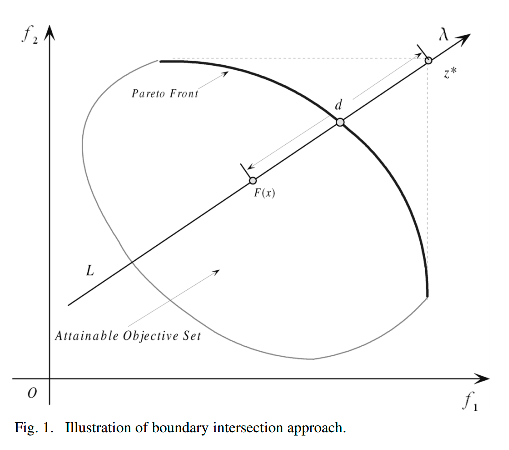m

i

n

g

b

i

(

x

∣

λ

,

z

∗

)

=

d

min \ g^{bi}(x|\lambda,z^*) = d

s

u

b

j

e

c

t

t

o

z

∗

−

F

(

x

)

=

d

λ

,

x

∈

Ω

subject\ to\ z^*-F(x) = d\lambda, \ x \in \varOmega

当

λ

\lambda

和

z

∗

z^*

分别是权重向量和参考点时，上图中约束

z

∗

−

F

(

x

)

=

d

λ

z^*-F(x)=d\lambda

保证

F

(

x

)

F(x)

这个点总是在方向为

λ

\lambda

且穿过

z

∗

z^*

的直线L上。优化的目标是尽可能高的将F(x)推至可达到目标集合的边界上。 ​  上述方法其中有个最大的缺点是处理相等约束。我们可以使用罚函数来解决约束问题，具体如下：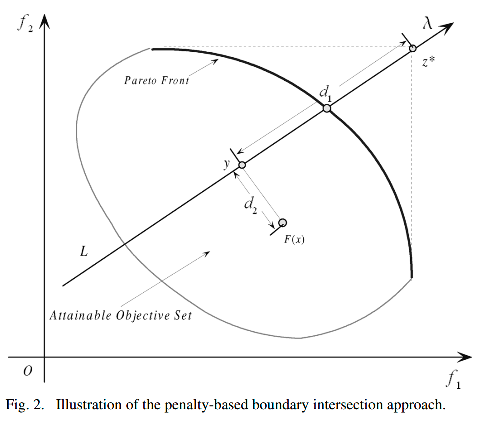m

i

n

g

b

i

p

(

x

∣

λ

,

z

∗

)

=

d

1

+

θ

d

2

min \ g^{bip}(x|\lambda,z^*)=d_1+\theta d_2

s

u

b

j

e

c

t

t

o

x

∈

Ω

subject \ to\ x \in \varOmega

where

d

1

=

∥

(

z

∗

−

F

(

x

)

)

T

λ

∥

∥

λ

∥

d_1 = \frac {\lVert (z^* - F(x))^T \lambda \rVert} {\lVert \lambda \rVert}

a

n

d

d

2

=

∥

F

(

x

)

−

(

z

∗

−

d

1

λ

)

∥

and \ d_2 = \lVert F(x) - (z^* - d_1\lambda) \rVert

θ

&gt;

0

\theta &gt; 0

是一个预设的惩罚参数。

y

y

是F(x)在直线L上的投影，

d

1

d_1

是

z

∗

z^*

和y的距离，

d

2

d_2

是F(x)和L的距离。当

θ

\theta

去适当的值时，两种边界交叉法的结果会非常接近。
MOEA/D的算法步骤(Tchebycheff Approach)
令

λ

j

=

(

λ

1

j

,

.

.

.

,

λ

m

j

)

T

\lambda^j = (\lambda_1^j,...,\lambda_m^j)^T

为一组均匀分布的权重向量，

z

∗

z^*

为参考点，第j个问题的目标函数为：

g

t

e

(

x

∣

λ

j

,

z

∗

)

=

max

⁡

1

≤

i

≤

m

{

λ

i

j

∣

f

i

(

x

)

−

z

i

∗

∣

}

g^te(x|\lambda^j,z^*)= \max_{1 \leq i \leq m}\{\lambda_i^j | f_i(x) - z_i^*|\}

MOEA/D可以在一次运行中，同时优化所有这N个子问题目标函数。 在MOEA/D中，权重向量的邻居中取几个最接近的权重向量，每一代的种群由每个子问题的最优解组成。只有相邻子问题可以进行优化当前的解。

YES

NO

开始

初始化种群

为每个子问题分配权重向量

运用遗传算子使相邻问题的最优解产生当前问题的解

根据聚合函数更新父代种群

满足终止条件

结束

每一代(t)，MOEA/D(Techebycheff)包括：

x

1

,

.

.

.

,

x

N

∈

Ω

x^1,...,x^N \in \varOmega

由N个点组织成的种群，

x

i

x^i

是第i个问题的当前解

F

V

i

,

.

.

.

,

F

V

N

FV^i,...,FV^N

,

F

V

i

=

F

(

x

i

)

FV^i = F(x^i)

z

=

(

z

1

,

.

.

.

,

z

m

)

T

​

z = (z_1 , ... ,z_m)^T​

,

z

i

z_i

是目标

f

i

f_i

中已发现最好的值。EP用来存储咋搜索过程中的非支配解
Input:
MOP(1);算法停止条件N：分解成的子问题的个数N个分布均匀的权重向量T：每个权重向量相邻的权重向量的个数 Output:EP
Step 1) 初始化： ​ Step 1.1) Set EP =

∅

\empty

。
​ Step 1.2) 计算任意两个权重向量的欧氏距离，找出每个权重向量最近邻的T个权重向量，对每一个

i

=

1

,

.

.

.

,

N

i = 1,...,N

,设$B(i) = {i_1,…,i_T}, 其 中 其中 \lambda{i1},…,\lambda{iT} 是 最 接 近 是最接近 \lambda^i$的N个权重向量。
​ Step 1.3) 随机或者使用特定方法生成一个初始化种群

x

1

,

.

.

.

,

x

N

x^1,...,x^N

。设

F

V

i

=

F

(

x

i

)

FV^i = F(x^i)

。
​ Step 1.4) 根据特定的问题初始化

z

=

(

z

1

,

.

.

.

,

z

m

)

T

z = (z_1,...,z_m)^T

。
Step 2) 更新： ​ 循环对

i

=

1

,

.

.

.

,

N

i=1,...,N

做以下操作 ​ Step 2.1)繁殖： 从

B

(

i

)

B(i)

中随机选择两个索引

k

,

l

k,l

，

x

k

x^k

和

x

l

x^l

使用遗传算子生成新的解

y

y

。
​ Step 2.2)改善： 根据特定问题，使用启发式方法改进

y

y

生成

y

′

y&#x27;

。
​ Step 2.3)更新z： 对于每一个$j = 1 , …,m ， 如 果 ，如果 z_j < f_i(y’) , 使 ,使 z_j = f_j(y’)$。
​ Step 2.4)更新邻域解： 对每个属于

B

(

i

)

B(i)

的索引j，如果

g

t

e

(

y

′

∣

λ

j

,

z

)

≤

g

t

e

(

x

j

∣

λ

j

,

z

)

g^{te}(y&#x27;|\lambda^j,z) \leq g^{te}(x^j|\lambda^j,z)

，则令

x

j

=

y

′

x^j = y&#x27;

，

F

V

j

=

F

(

y

′

)

FV^j = F(y&#x27;)

。
​ Step 2.5)更新EP： ​ 1.删除EP中所有被

F

(

y

′

)

F(y&#x27;)

支配的向量。 ​ 2.如果EP中没有向量支配

F

(

y

′

)

F(y&#x27;)

，则将

F

(

y

′

)

​

F(y&#x27;)​

加入到EP中。
Step 3)停止条件： 如果满足停止条件，停止并且输出EP，否则则跳转至Step 2。
MOEA/D与NSGA-II的对比
评价准则
1.1 C-Metric ​ A和B是MOP问题的两个接近的PF集合

C

(

A

,

B

)

=

∣

{

u

∈

B

∣

∃

v

∈

A

:

v

d

o

m

i

n

a

t

e

s

u

}

∣

∣

B

∣

C(A,B) = \frac {\lvert \{u \in B | \exist v \in A: v dominates \ u \} \rvert} {\lvert B \rvert}

C

(

A

,

B

)

C(A,B)

不等于

1

−

C

(

B

,

A

)

1-C(B,A)

。

C

(

A

,

B

)

=

1

C(A,B) = 1

表示B中所有的解都被A中的一些解支配。当

C

(

A

,

B

)

=

0

C(A,B)=0

表示B中没有解被A中支配。 1.2 D-Metric ​ 令

P

∗

P^*

为沿着PF的一组分布均匀的点，令A为接近PF的集合，

P

∗

P^*

和A的平均距离定义如下：

D

(

A

,

P

∗

)

=

∑

v

∈

P

∗

d

(

v

,

A

)

∣

P

∗

∣

D(A,P^*) = \frac {\displaystyle\sum_{v \in P^*}d(v,A)} {\lvert P^* \rvert}

d

(

v

,

A

)

d(v,A)

是

v

v

和A中的点之间的最小欧氏距离。如果

∣

P

∗

∣

​

\lvert P^* \rvert​

足够大，说明集合很好的代表了PF，

D

(

A

,

P

∗

)

D(A,P^*)

可以一定程度上评估A的多样性和收敛性。
MOEA/D与NSGA-II的对比
2.1 时间复杂度对比
​ 采用改良的MOEA/D进行实验，去掉了额外种群EP,因此去掉步骤2.5，去掉了启发式优化过程，因此删去了步骤2.2，所以时间复杂度主要在步骤2部分。步骤2.3进行了

O

(

m

)

O(m)

次比较，步骤2.4部分的时间复杂度为

O

(

m

T

)

O(mT)

，由于分成了N个子问题，所以改良的MOEA/D的时间复杂度为

O

(

m

T

N

)

O(mTN)

。而NSGA-II的时间复杂度为

O

(

m

N

2

)

O(mN^2)

。因为N为权重向量的个数，而T为一个权重向量相邻的权重向量的个数，所以

T

&lt;

N

T &lt; N

，故而MOEA/D的时间复杂度比NSGA-II要小，但时间复杂度的优势不是非常明显。 2.2 空间复杂度对比
​ 改良后的MOEA/D没有EP，只有m个参照点z需要存储，但是与一代种群大小相比，所占空间非常小，我觉得两者是空间复杂度上没有特别大的差别。
2.3 测试函数及实验结果 根据论文中，针对2个目标问题将NSGA-II和MOEA/D的种群大小都100。针对3目标问题，种群数都设为300，都采用模拟二进制的交叉变异和多项式变异，

p

c

=

1.0

,

p

m

=

1

/

n

,

T

=

20

p_c=1.0,p_m=1/n,T=20

ZDT1

f

1

(

X

)

=

x

1

f_1(X) = x_1

f

2

(

X

)

=

g

(

X

)

[

1

−

x

1

/

g

(

X

)

]

f_2(X) = g(X)[1-\sqrt{x_1/g(X)}]

g

(

X

)

=

1

+

9

(

∑

i

=

2

n

x

i

)

/

(

n

−

1

)

g(X) = 1+9(\sum_{i=2}^nx_i)/(n-1)

n

=

30

,

n=30,

x

1

∈

[

0

,

1

]

,

x

i

=

0

x_1\in[0,1],x_i = 0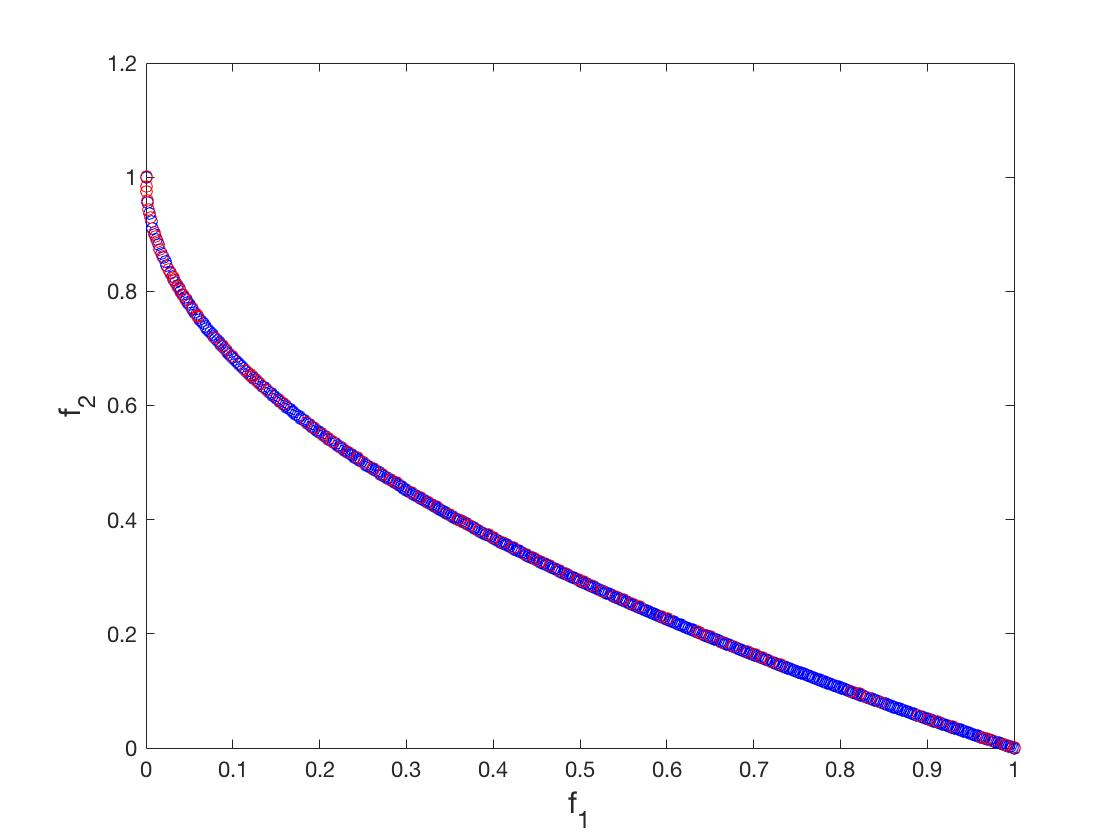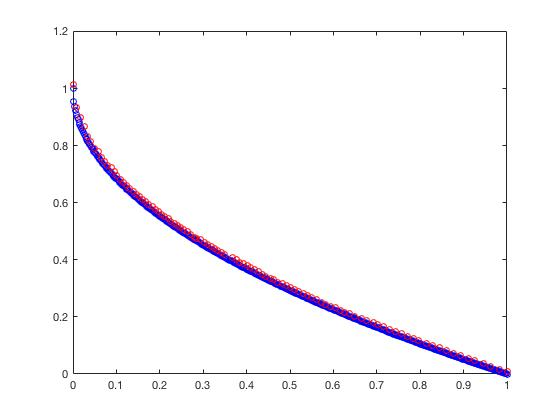NSGA-II                MOEA/D
ZDT2

f

1

(

X

)

=

x

1

f_1(X) = x_1

f

2

(

X

)

=

g

(

X

)

[

1

−

(

x

1

/

g

(

X

)

)

2

]

f_2(X) = g(X)[1-(x_1/g(X))^2]

g

(

X

)

=

1

+

9

(

∑

i

=

2

n

x

i

)

/

(

n

−

1

)

g(X) = 1+9(\sum_{i=2}^nx_i)/(n-1)

n

=

30

,

n=30,

x

1

∈

[

0

,

1

]

,

x

i

=

0

x_1\in[0,1],x_i = 0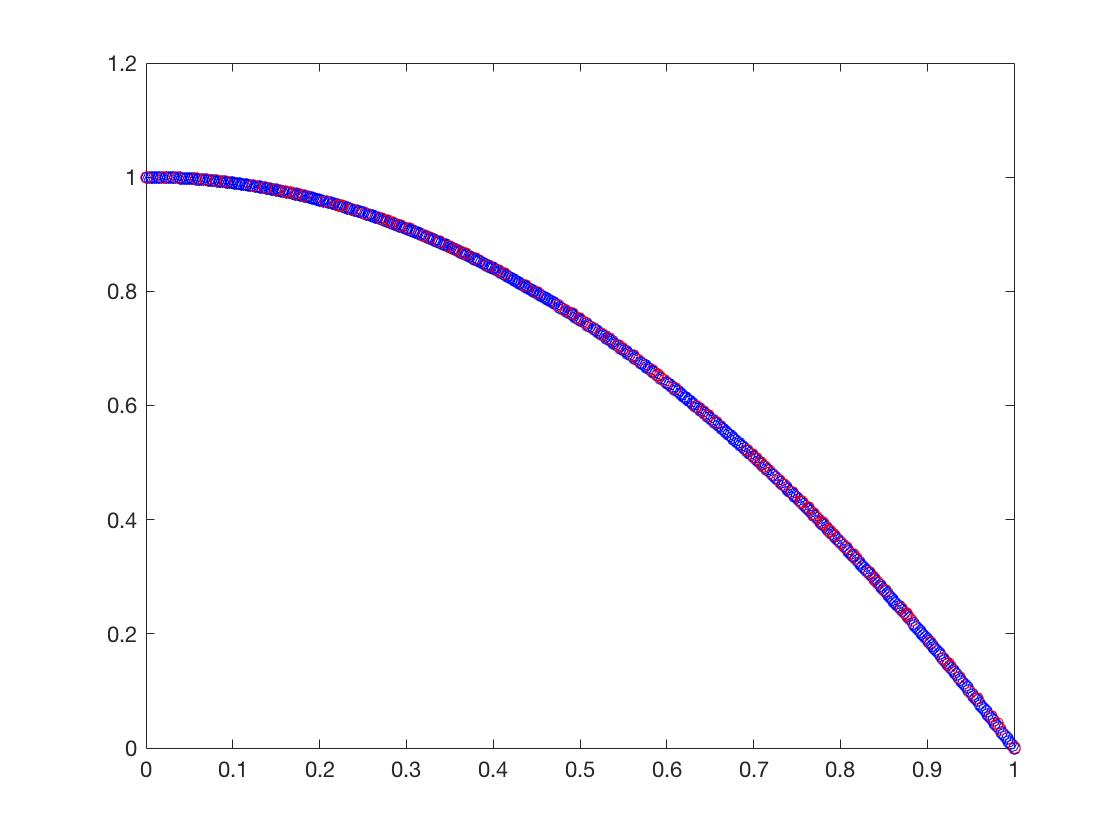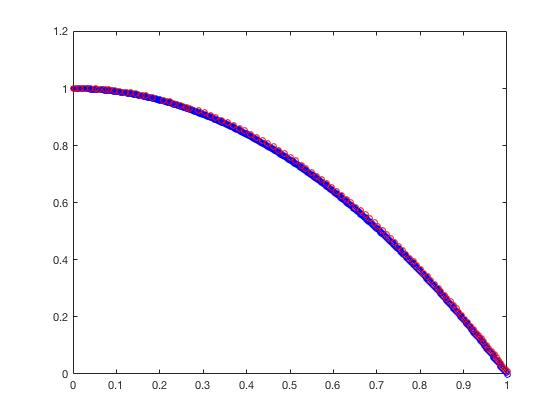NSGA-II                MOEA/D
ZDT3

f

1

(

X

)

=

x

1

f_1(X) = x_1

f

2

(

X

)

=

g

(

X

)

[

1

−

x

1

/

g

(

X

)

−

x

1

g

(

X

)

sin

⁡

(

10

π

x

1

)

]

f_2(X) = g(X)[1-\sqrt{x_1/g(X)}-{\frac{x_1}{g(X)}}\sin(10\pi x_1)]

g

(

X

)

=

1

+

9

(

∑

i

=

2

n

x

i

)

/

(

n

−

1

)

g(X) = 1+9(\sum_{i=2}^nx_i)/(n-1)

n

=

30

,

n=30,

x

1

∈

[

0

,

1

]

,

x

i

=

0

x_1\in[0,1],x_i = 0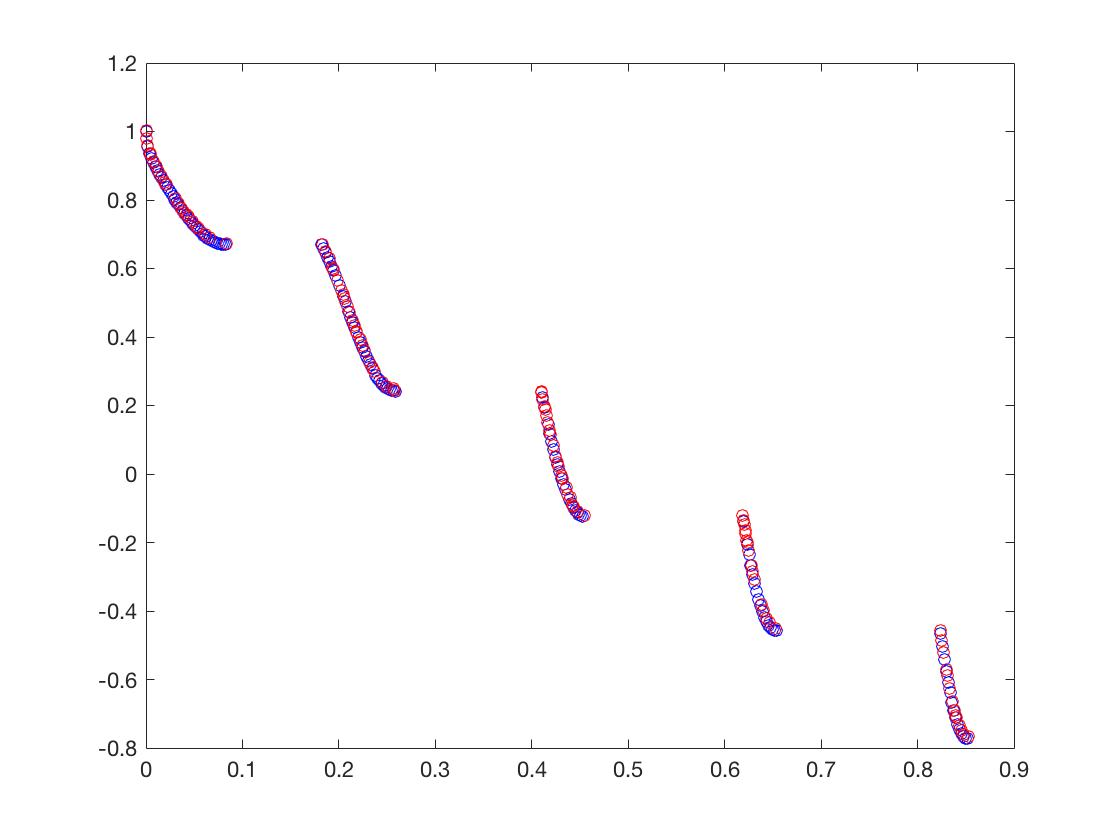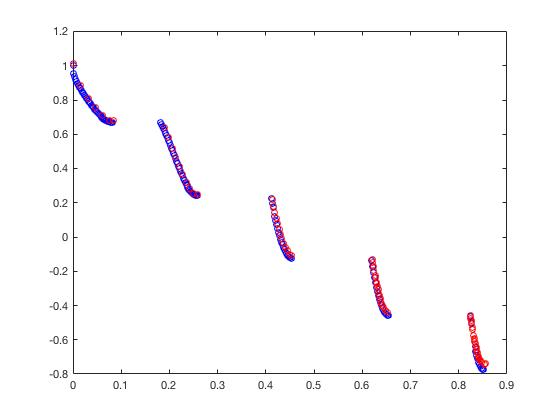NSGA-II                MOEA/D
ZDT4

f

1

(

X

)

=

x

1

f_1(X) = x_1

f

2

(

X

)

=

g

(

X

)

[

1

−

x

1

/

g

(

X

)

]

f_2(X) = g(X)[1-\sqrt{x_1/g(X)}]

g

(

X

)

=

1

+

10

(

n

−

1

)

+

∑

i

=

2

n

[

x

i

2

−

10

cos

⁡

(

4

π

x

i

)

]

g(X) = 1+10(n-1)+\sum_{i=2}^n[x_i^2-10\cos(4\pi x_i)]

n

=

10

,

n=10,

x

1

∈

[

0

,

1

]

,

x

i

=

0

x_1\in[0,1],x_i = 0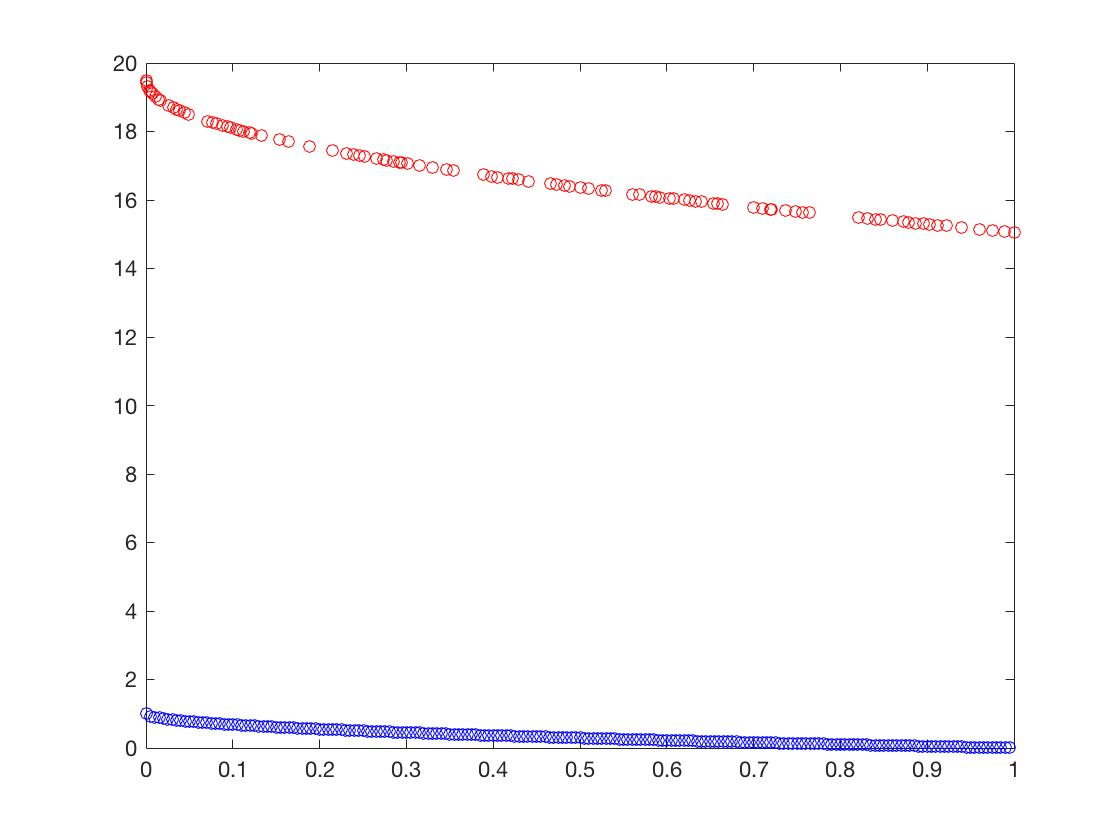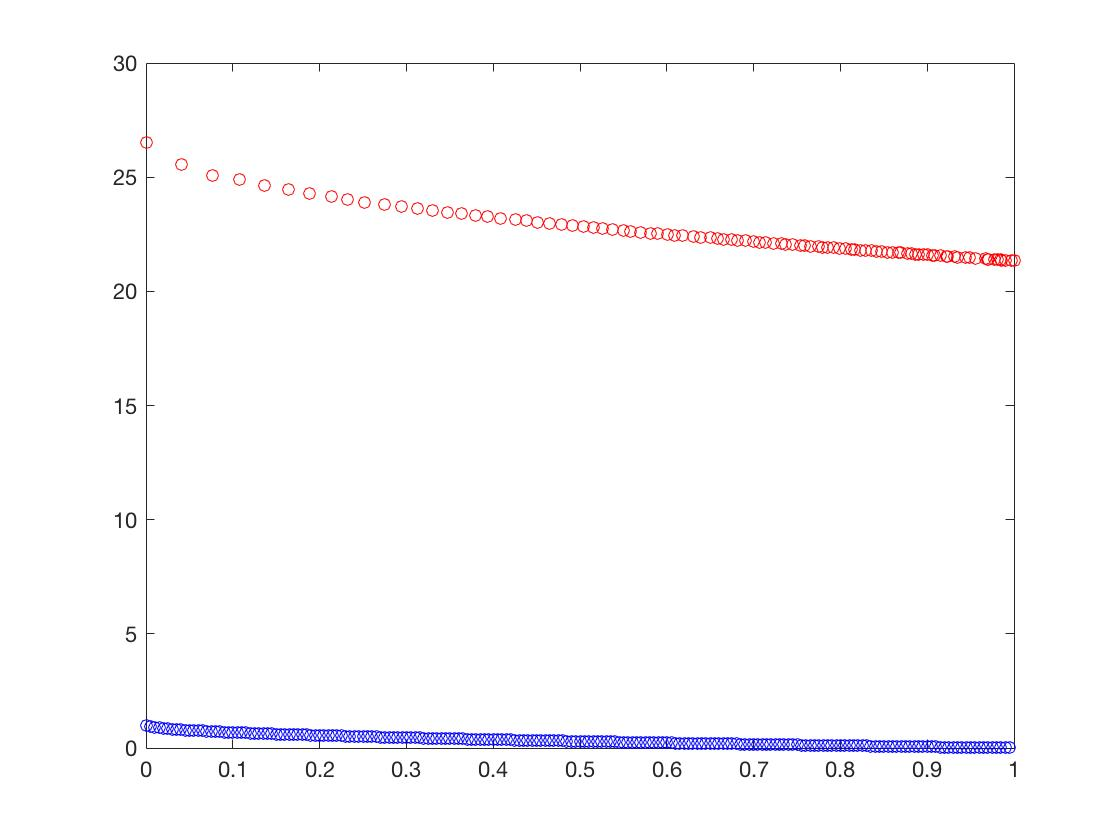NSGA-II                MOEA/D
ZDT6

f

1

(

X

)

=

1

−

e

x

p

(

−

4

π

x

1

)

sin

⁡

6

(

6

π

x

1

)

f_1(X)= 1-exp(-4\pi x_1)\sin^6(6\pi x_1)

f

2

(

X

)

=

g

(

X

)

[

1

−

(

f

1

(

X

)

/

g

(

X

)

)

2

]

f_2(X)= g(X)[1-(f_1(X)/g(X))^2]

g

(

X

)

=

1

+

9

[

(

∑

n

i

=

2

x

i

)

/

(

n

−

1

)

]

0.25

g(X) = 1+9[(\sum_n^{i=2}x_i)/(n-1)]^{0.25}

n

=

10

,

n=10,

x

1

∈

[

0

,

1

]

,

x

i

=

0

x_1\in[0,1],x_i = 0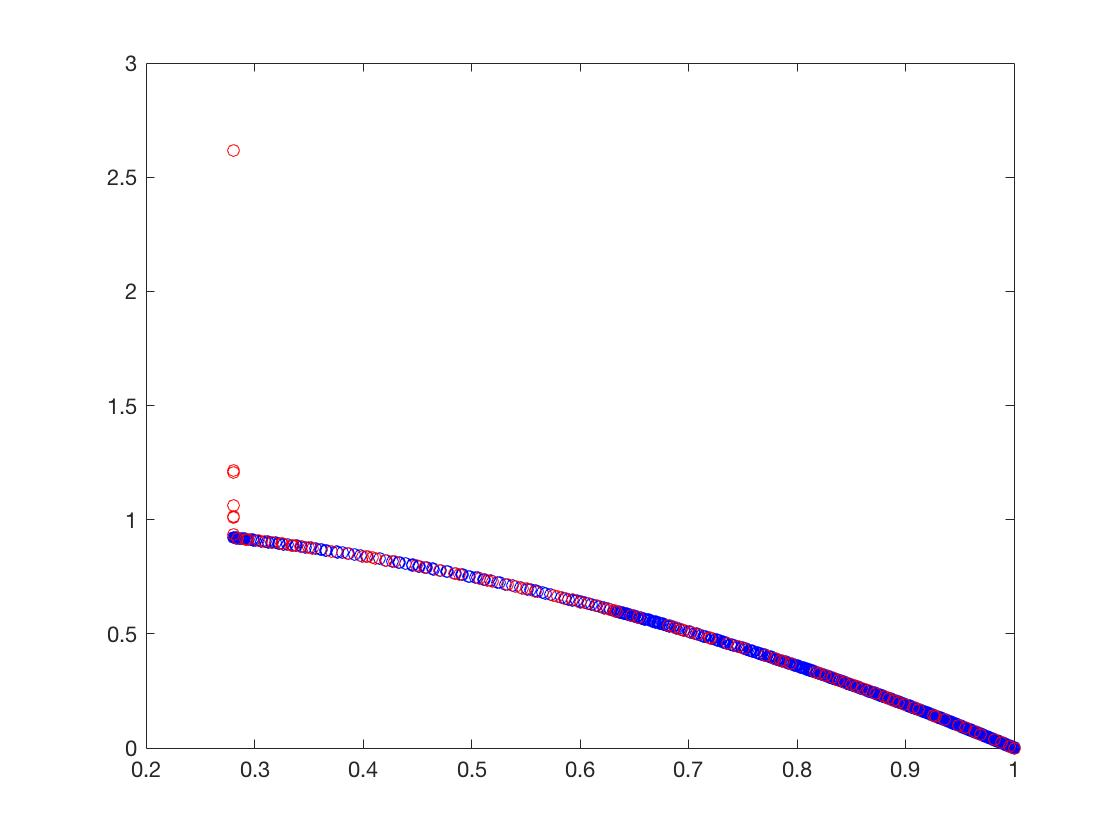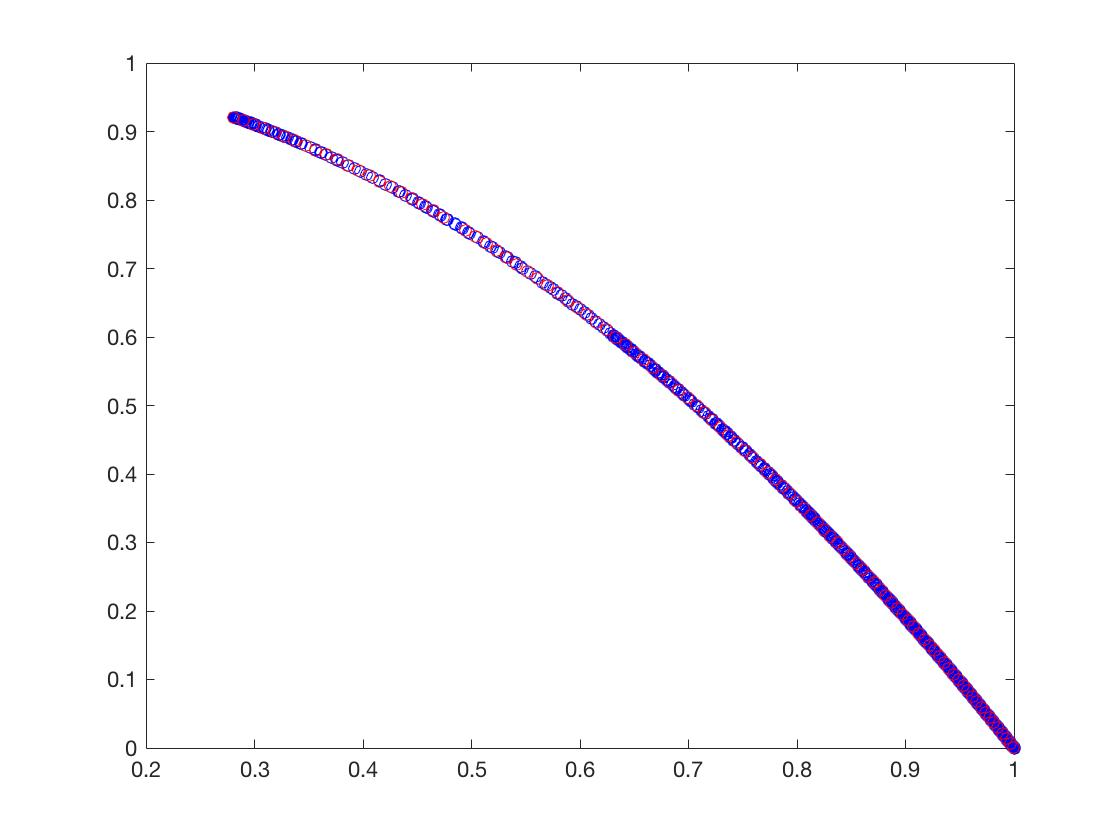NSGA-II                MOEA/D
DTLZ1

f

1

(

X

)

=

(

1

+

g

(

X

)

)

x

1

x

2

f_1(X) = (1+g(X))x_1x_2

f

2

(

X

)

=

(

1

+

g

(

X

)

)

x

1

(

1

−

x

2

)

f_2(X) = (1+g(X))x_1(1-x_2)

f

3

(

X

)

=

(

1

+

g

(

X

)

)

(

1

−

x

1

)

f_3(X) = (1+g(X))(1-x_1)

g

(

X

)

=

100

(

n

−

2

)

+

100

∑

i

=

3

n

{

(

x

i

−

0.5

)

2

−

cos

⁡

[

20

π

(

x

i

−

0.5

)

]

}

g(X) = 100(n-2)+100\sum_{i=3}^n\{(x_i-0.5)^2-\cos[20\pi(x_i-0.5)]\}

x

=

(

x

1

,

.

.

.

,

x

n

)

T

∈

[

0

,

1

]

n

x = (x_1,...,x_n)^T \in [0,1]^n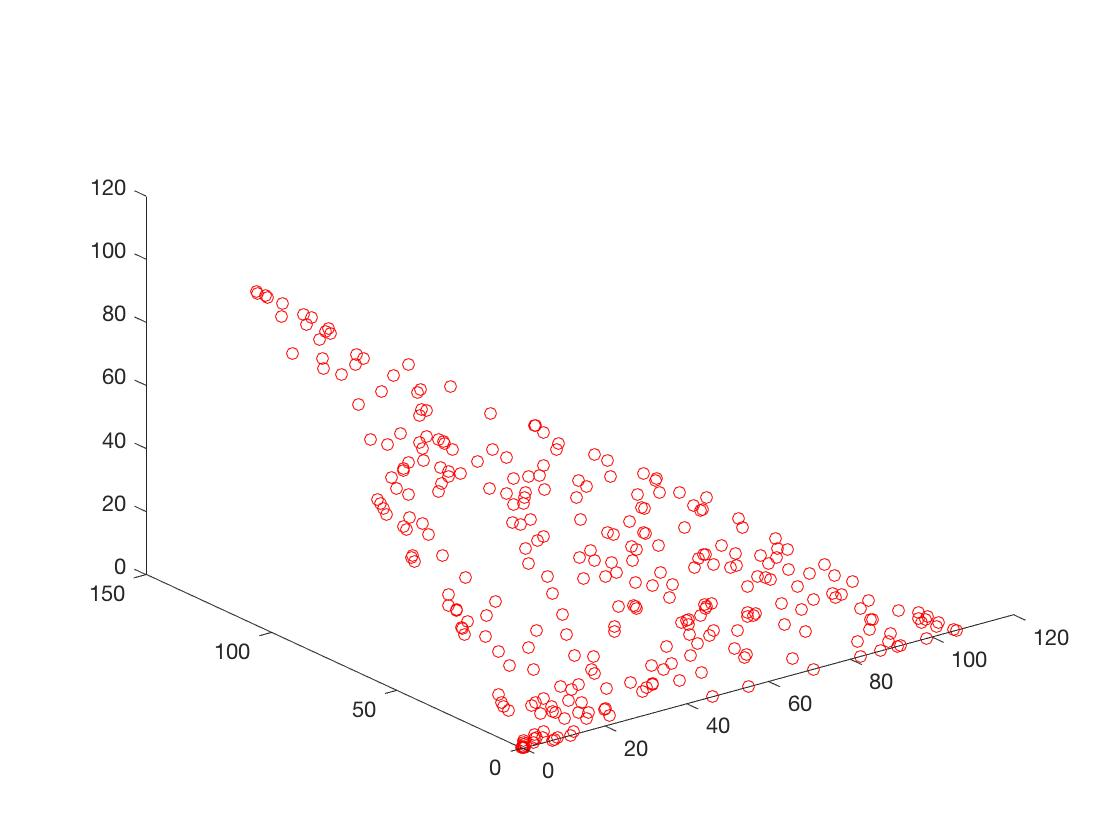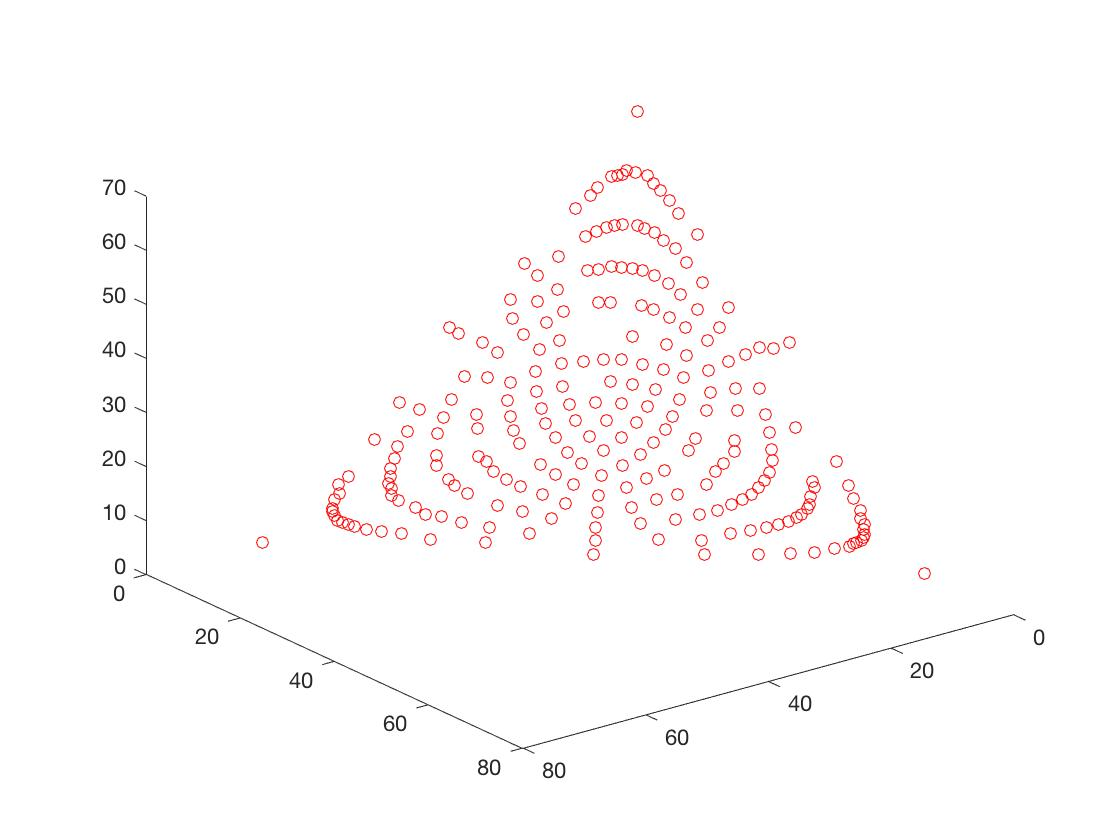NSGA-II                MOEA/D
DTLZ2

f

1

(

X

)

=

(

1

+

g

(

X

)

)

cos

⁡

(

x

1

π

2

)

cos

⁡

(

x

2

π

2

)

f_1(X) = (1+g(X))\cos(\frac {x_1\pi} {2})\cos(\frac {x_2\pi} {2})

f

2

(

X

)

=

(

1

+

g

(

X

)

)

cos

⁡

(

x

1

π

2

)

sin

⁡

(

x

2

π

2

)

f_2(X) = (1+g(X))\cos(\frac {x_1\pi} {2})\sin(\frac {x_2\pi} {2})

f

3

(

X

)

=

(

1

+

g

(

X

)

)

sin

⁡

(

x

1

π

2

)

f_3(X) = (1+g(X))\sin(\frac {x_1\pi} {2})

g

(

X

)

=

∑

i

=

3

n

x

i

2

g(X)=\sum_{i=3}^nx_i^2

x

=

(

x

1

,

.

.

.

,

x

2

)

T

∈

[

0

,

1

]

2

∗

[

−

1

,

1

]

n

−

2

x = (x_1,...,x_2)^T \in [0,1]^2*[-1,1]^{n-2}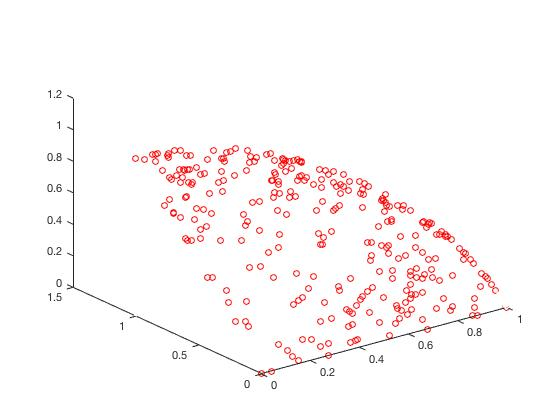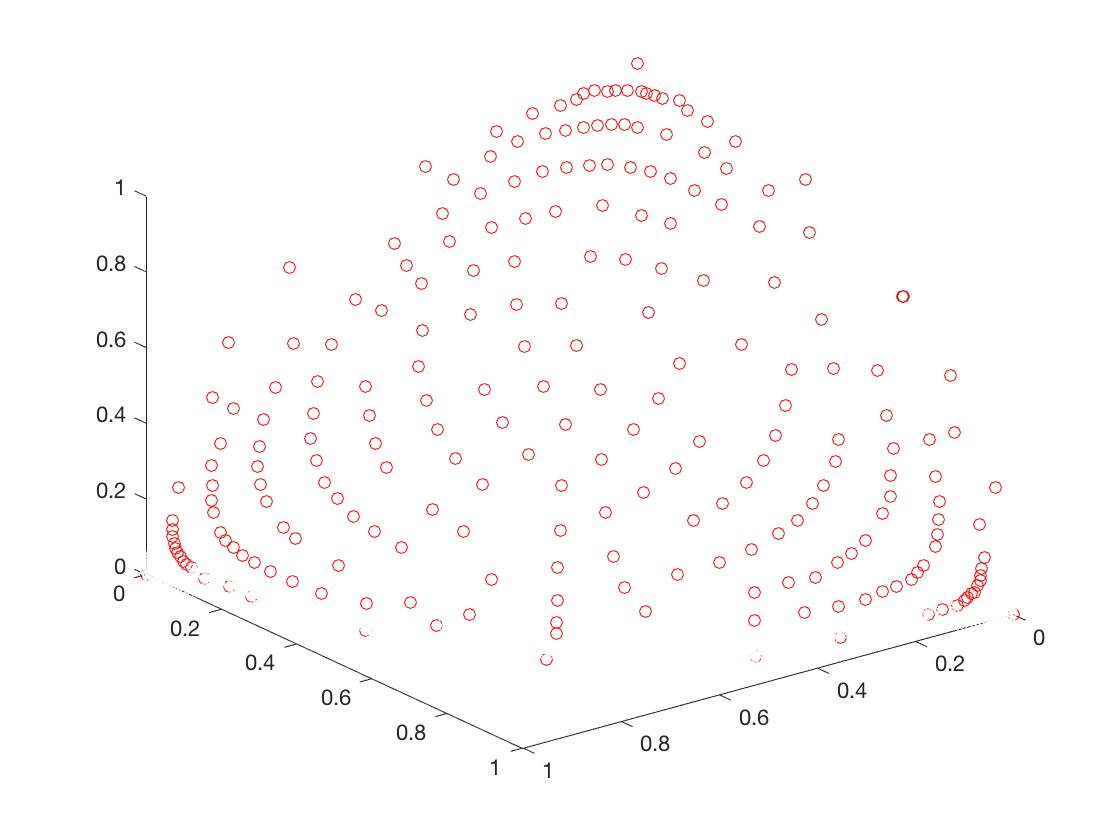NSGA-II                MOEA/D

Δ

\Delta

的Mean
ProblemZDT1ZDT2ZDT3ZDT4ZDT6DTLZ1DTLZ2NSGA-II0.6420.7060.4251.000.6100.9450.0323MOEA/D1.001.000.9311.000.0410.8300.0281

γ

\gamma

的Mean
ProblemZDT1ZDT2ZDT3ZDT4ZDT6DTLZ1DTLZ2NSGA-II0.005740.06620.0061429.2990.0039772.5580.07819MOEA/D0.01770.40170.013515.6720.0063544.7260.4634
可以从图的对比和表中

Δ

\Delta

与

γ

\gamma

两个参数的对比看出，在两目标问题上，两者算法相差不多，甚至在ZDT1、ZDT2、ZDT3等问题上NSGA-II更优一些，但是在ZDT6上MOEA/D表现的更好，MOEA/D的主要优势在算法的计算速度上，在面对三目标问题时，MOEA/D比NSGA-II要优秀很多。
展开全文NSGA-II
• Matlab 多目标优化 遗传算法 源程序 很好的应用案例 基于MATLABmatlab
• A structure MATLAB implementation of MOPSO for Evolutionary Multi-Objective Optimization A structured MATLAB implementation of MOEA/D for Evolutionary Multi-Objective Optimization...